# West Bengal Madhyamik Board Question Paper for Class 10th Maths 2020 In PDF

West Bengal Madhyamik (Class 10) Maths 2020 question paper with solutions are provided on this page by BYJU’S, in downloadable pdf format and also in the text, so that the students can solve the WBBSE Madhyamik maths question paper 2020 for better preparation of the West Bengal Madhyamik exam. Students can refer to the West Bengal Madhyamik (Class 10) Maths 2020 question paper to gauge their exam preparation level.

Maths Question Paper 2020 Class 10 is also added here, which assists in the exam preparation within the stipulated time. Students are able to access all the WBBSE madhyamik previous year maths question papers. Students can assess their preparation and confidence level by practising different types of questions in the previous year papers and also get acquainted with the paper pattern that one can expect for the board exams.

### WBBSE Class 10th Maths Question Paper With Solutions 2020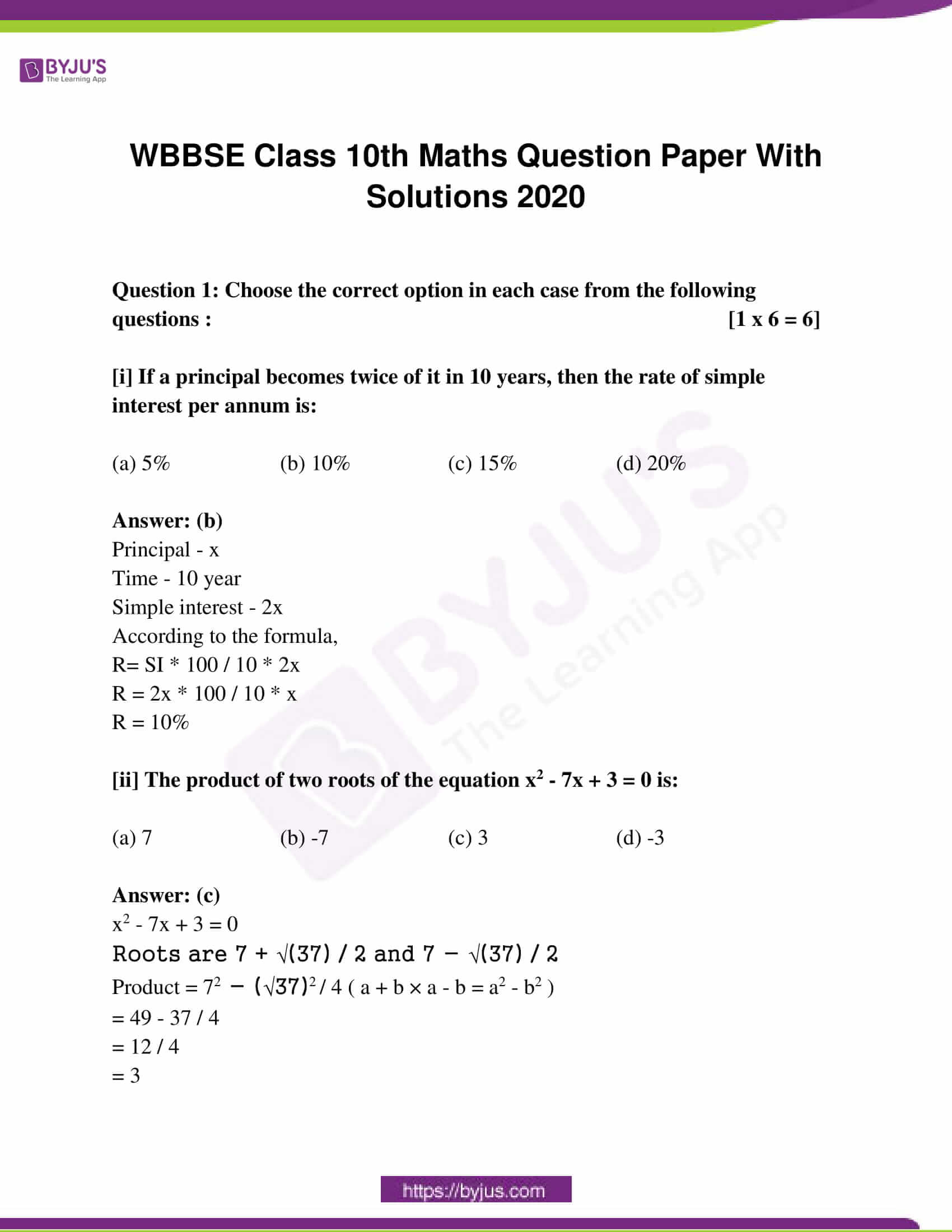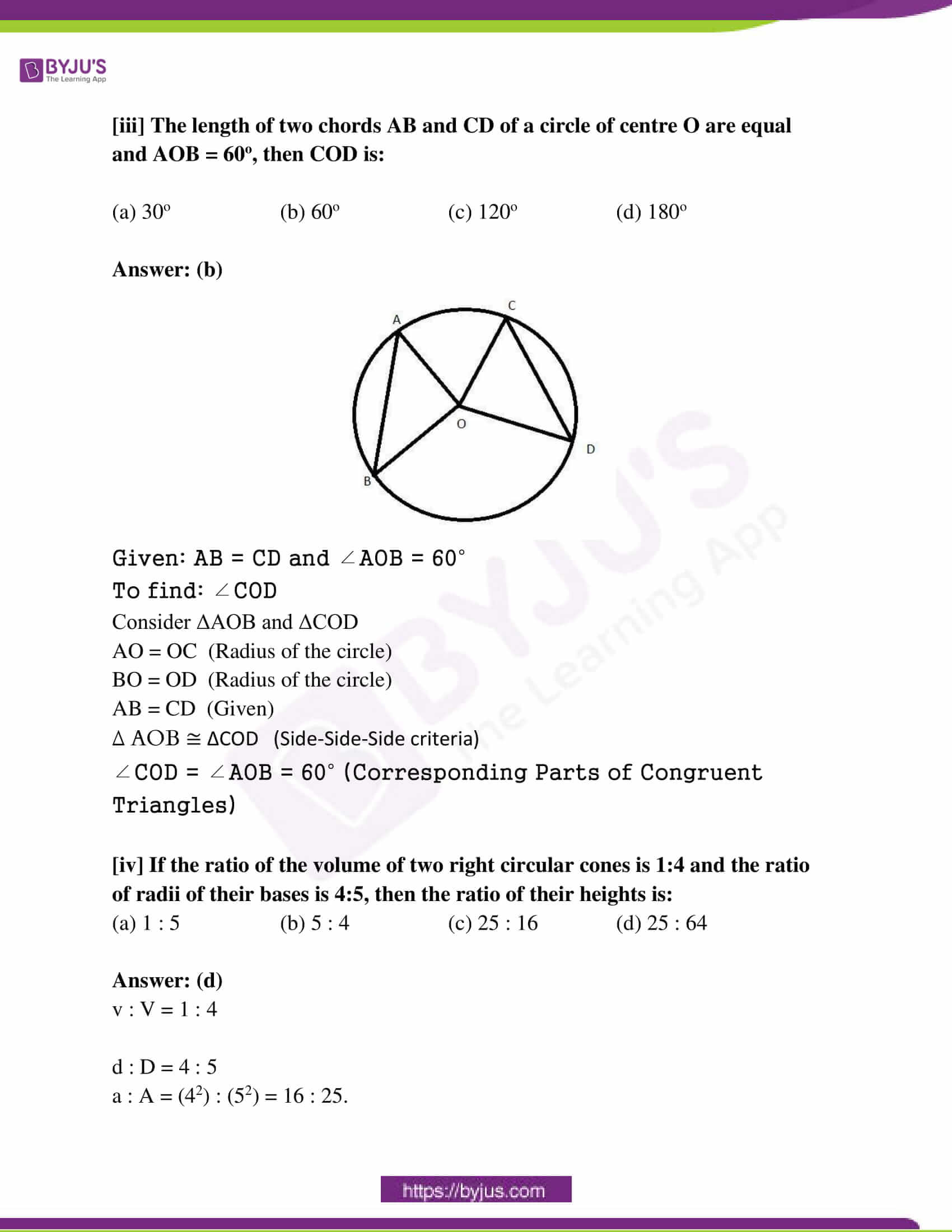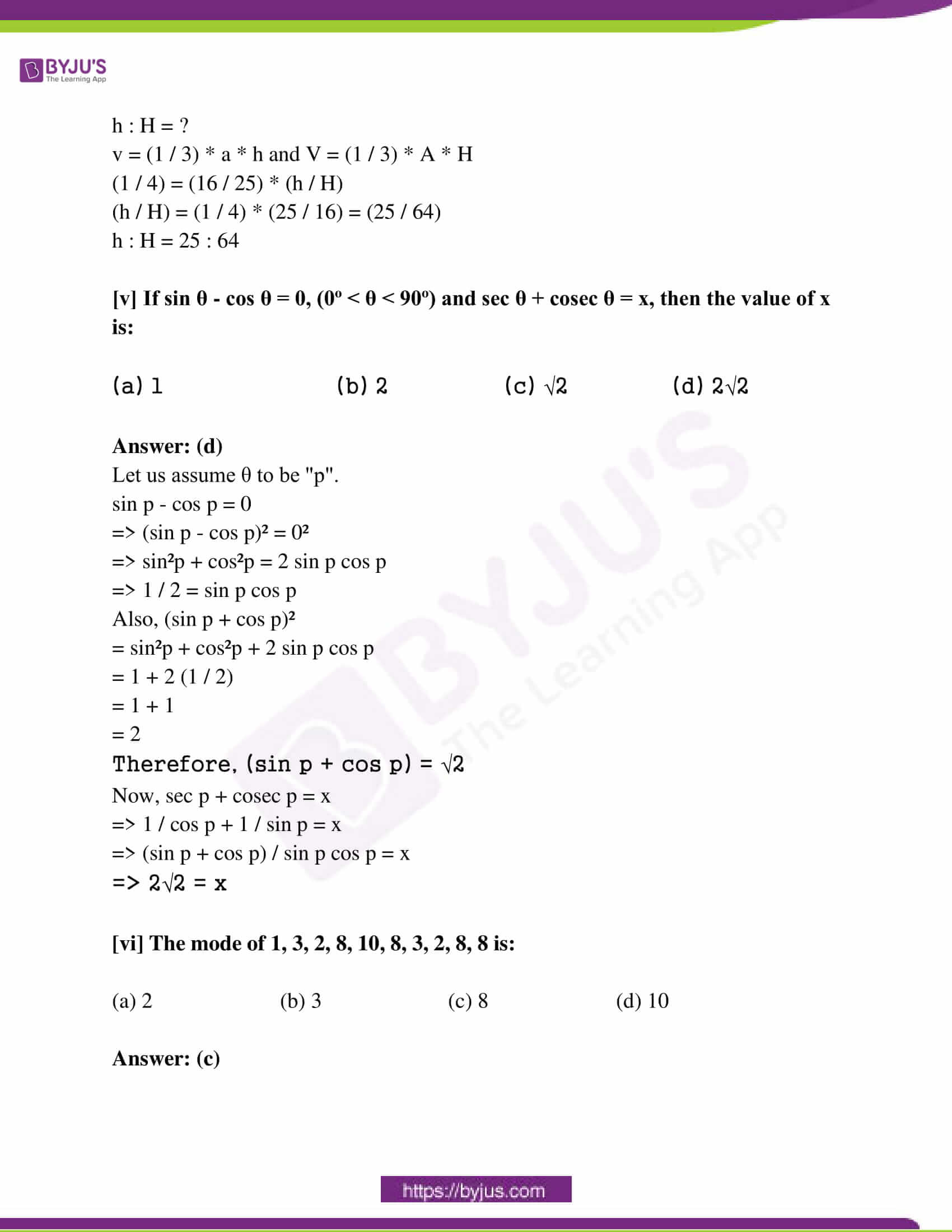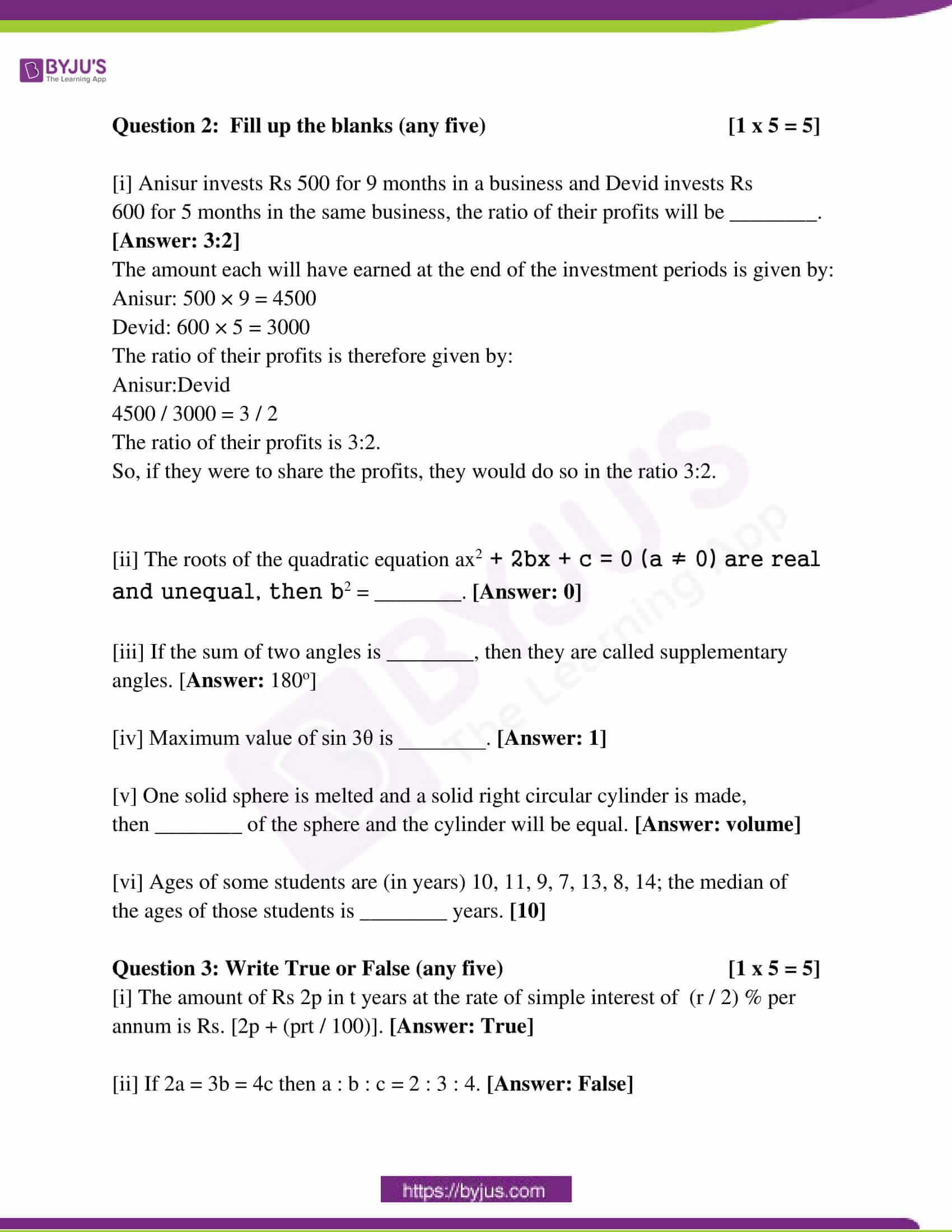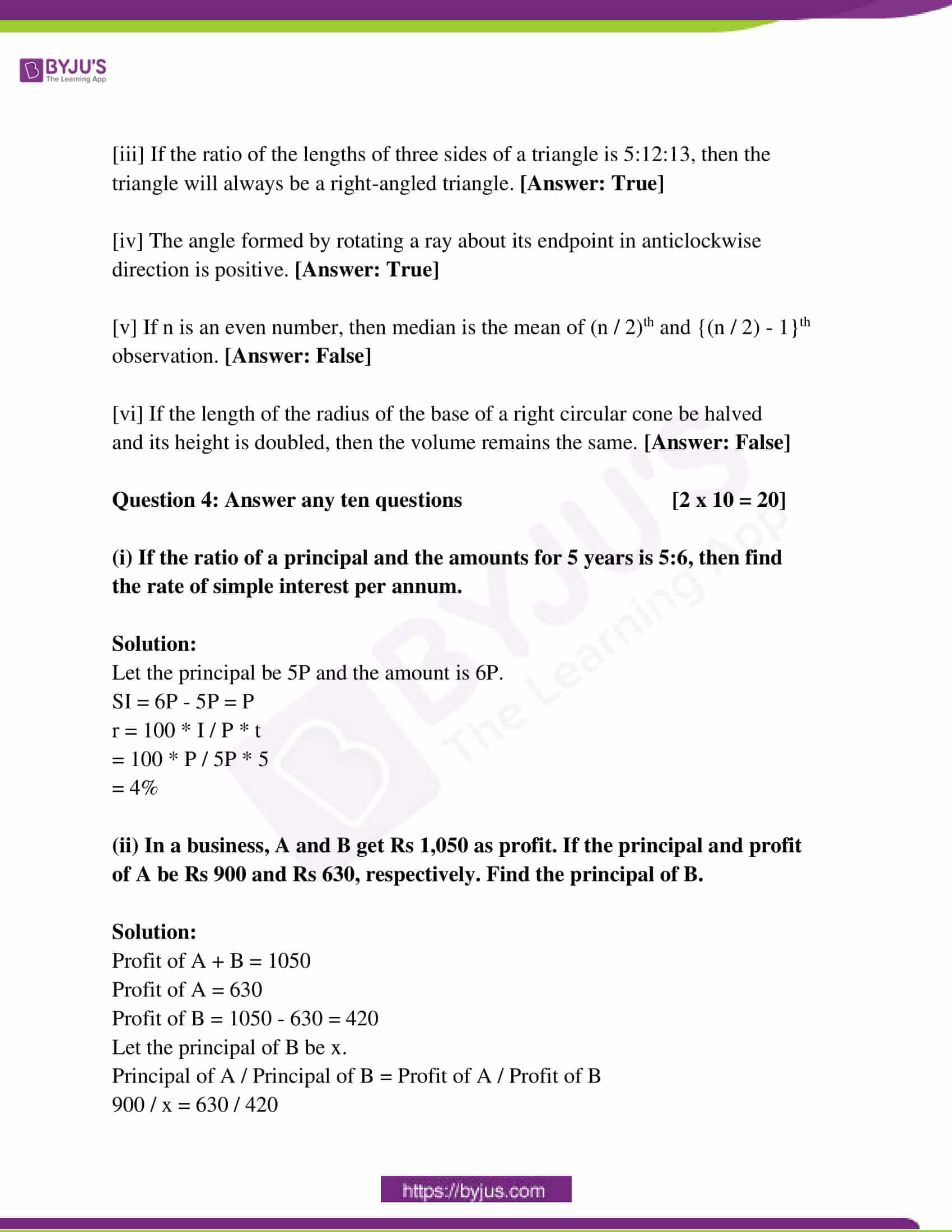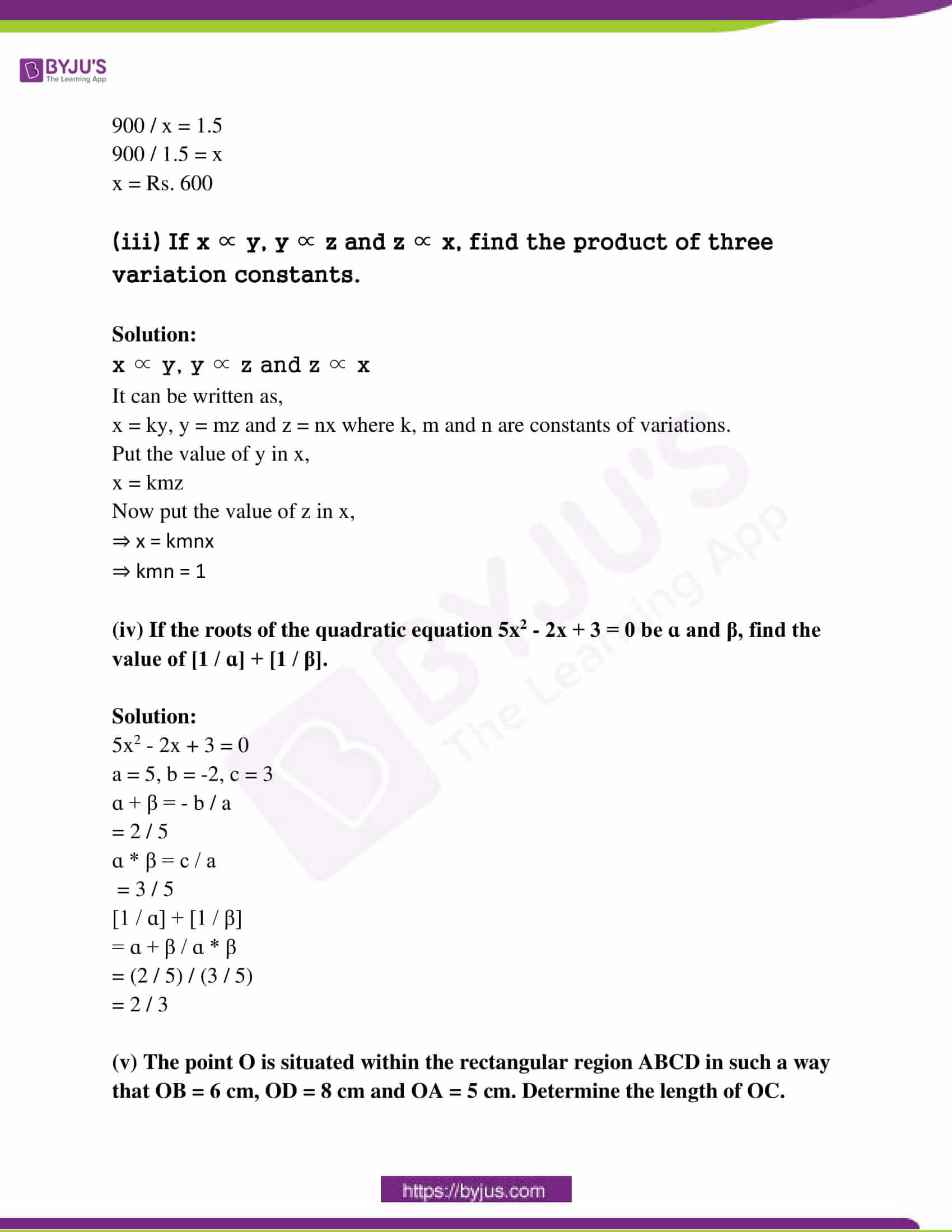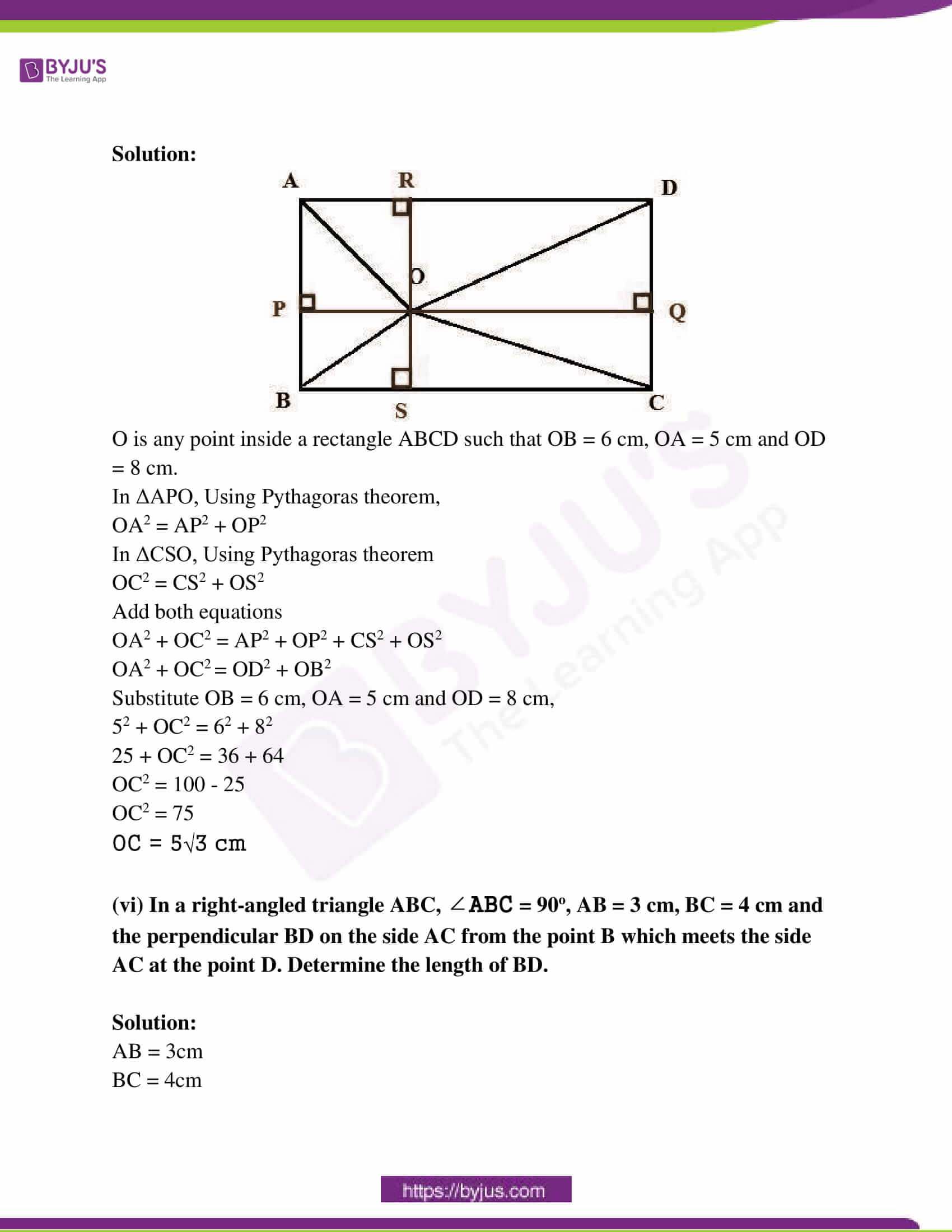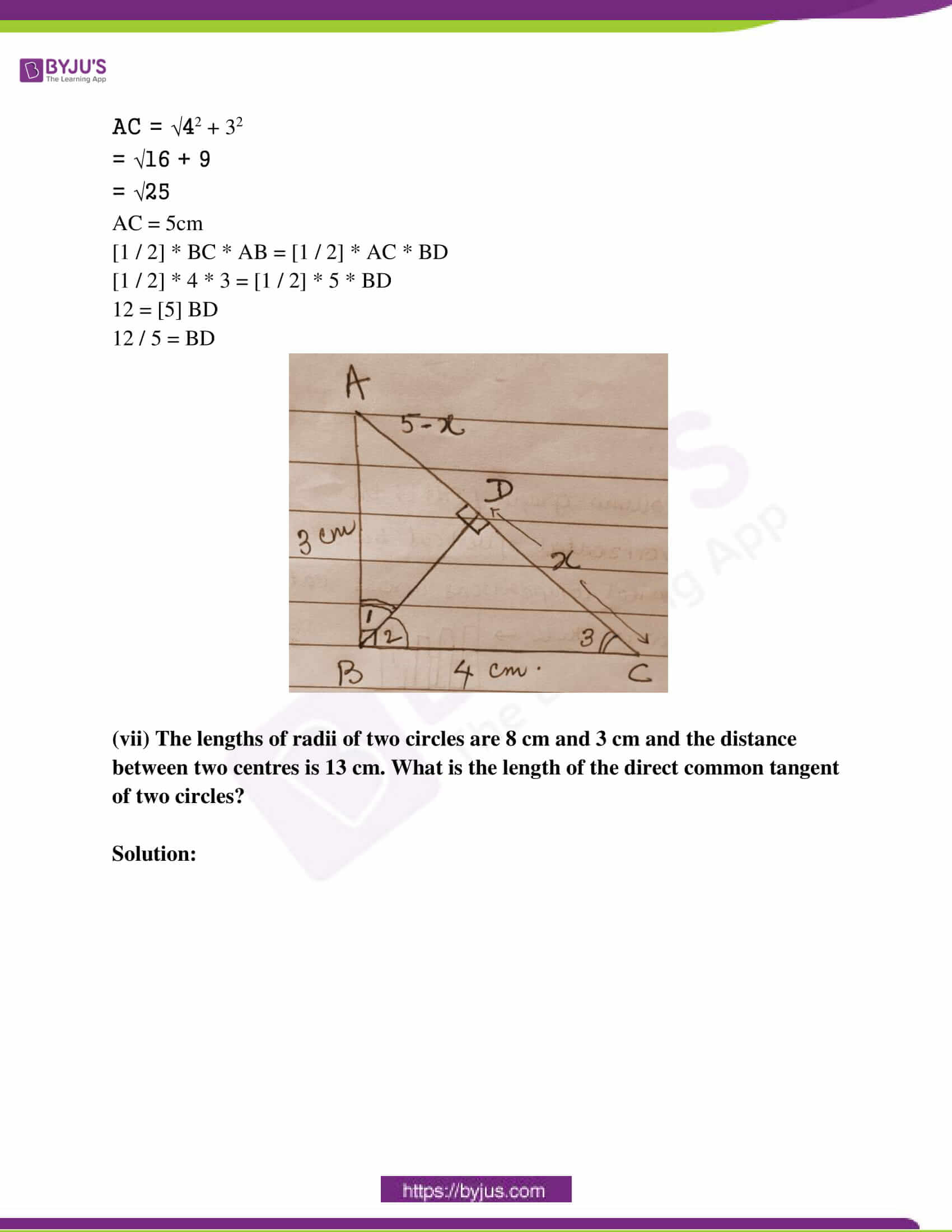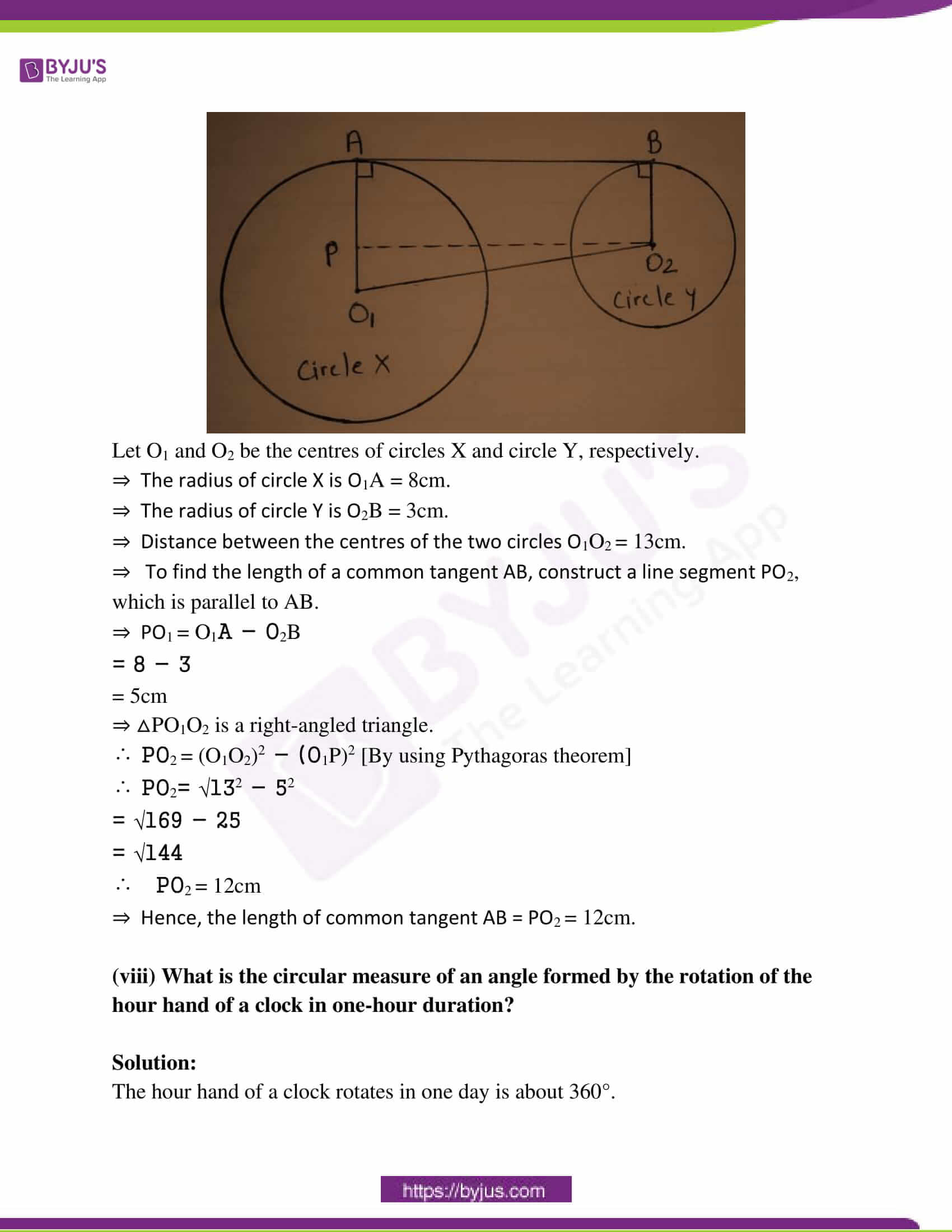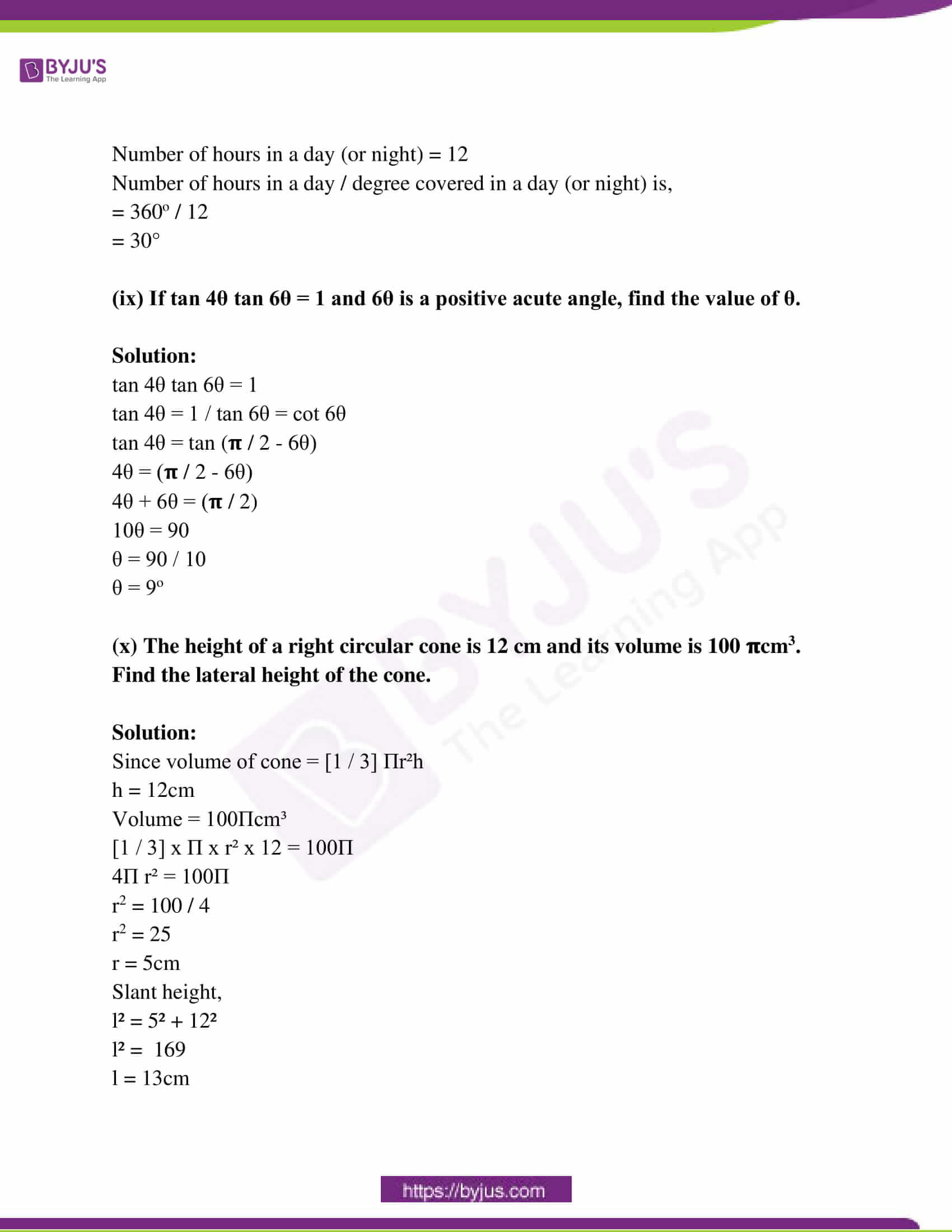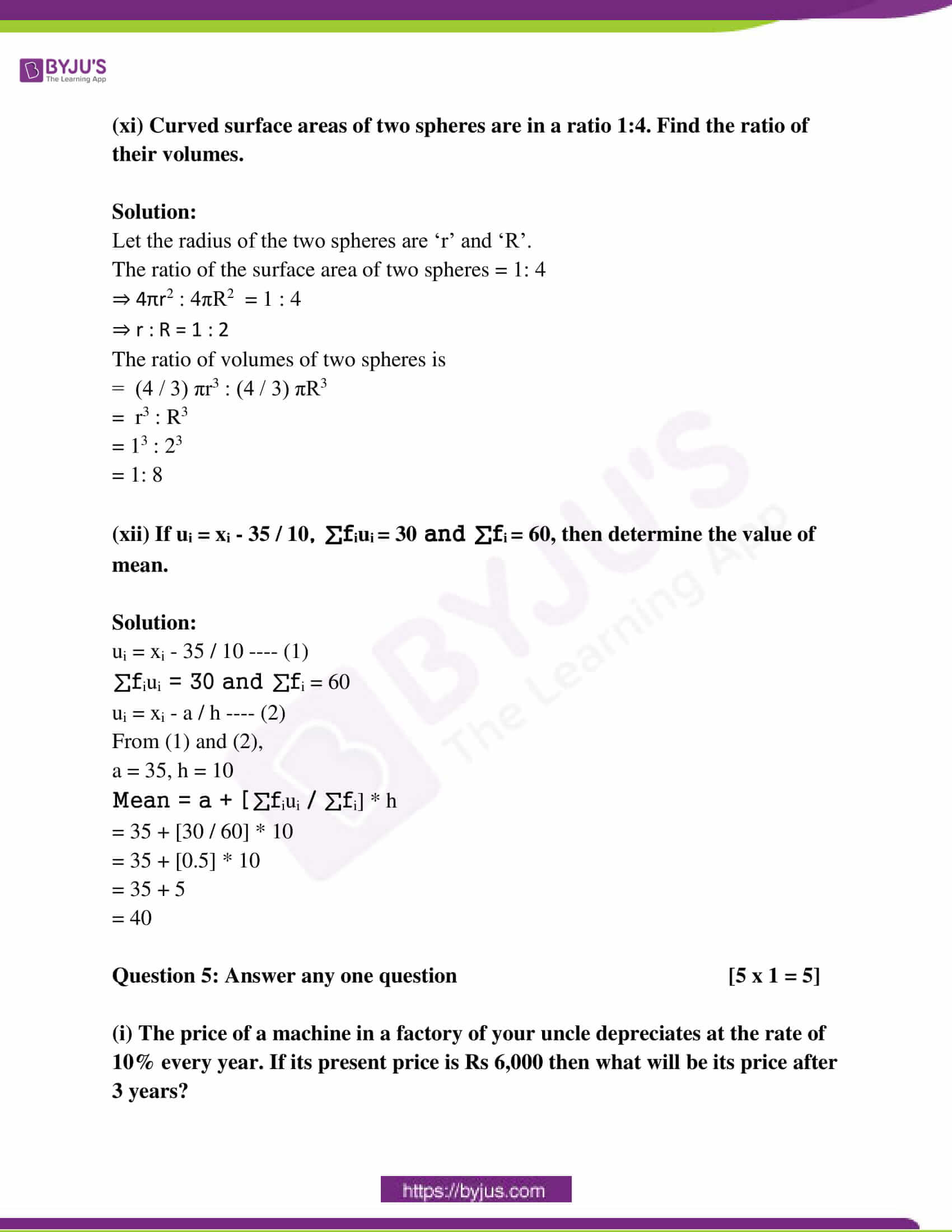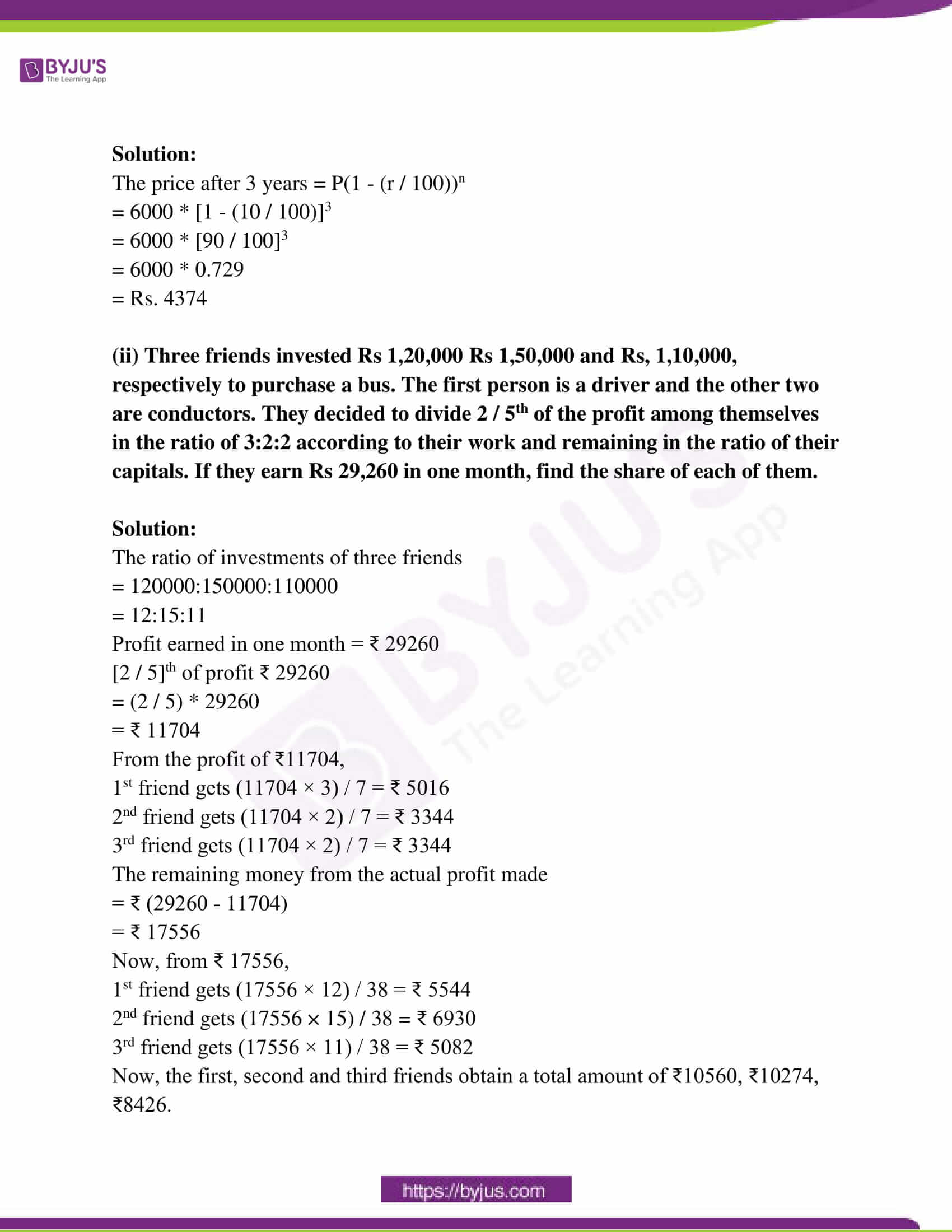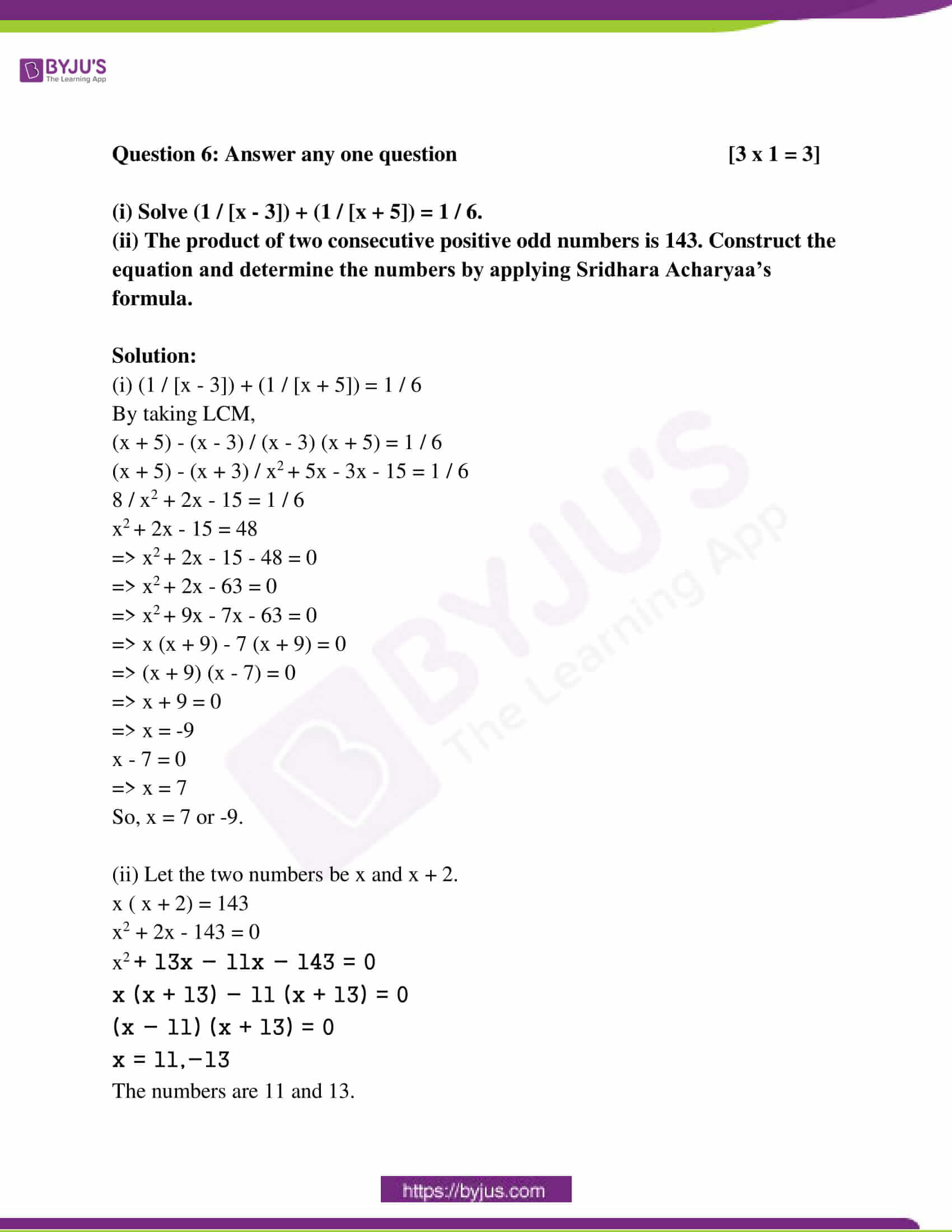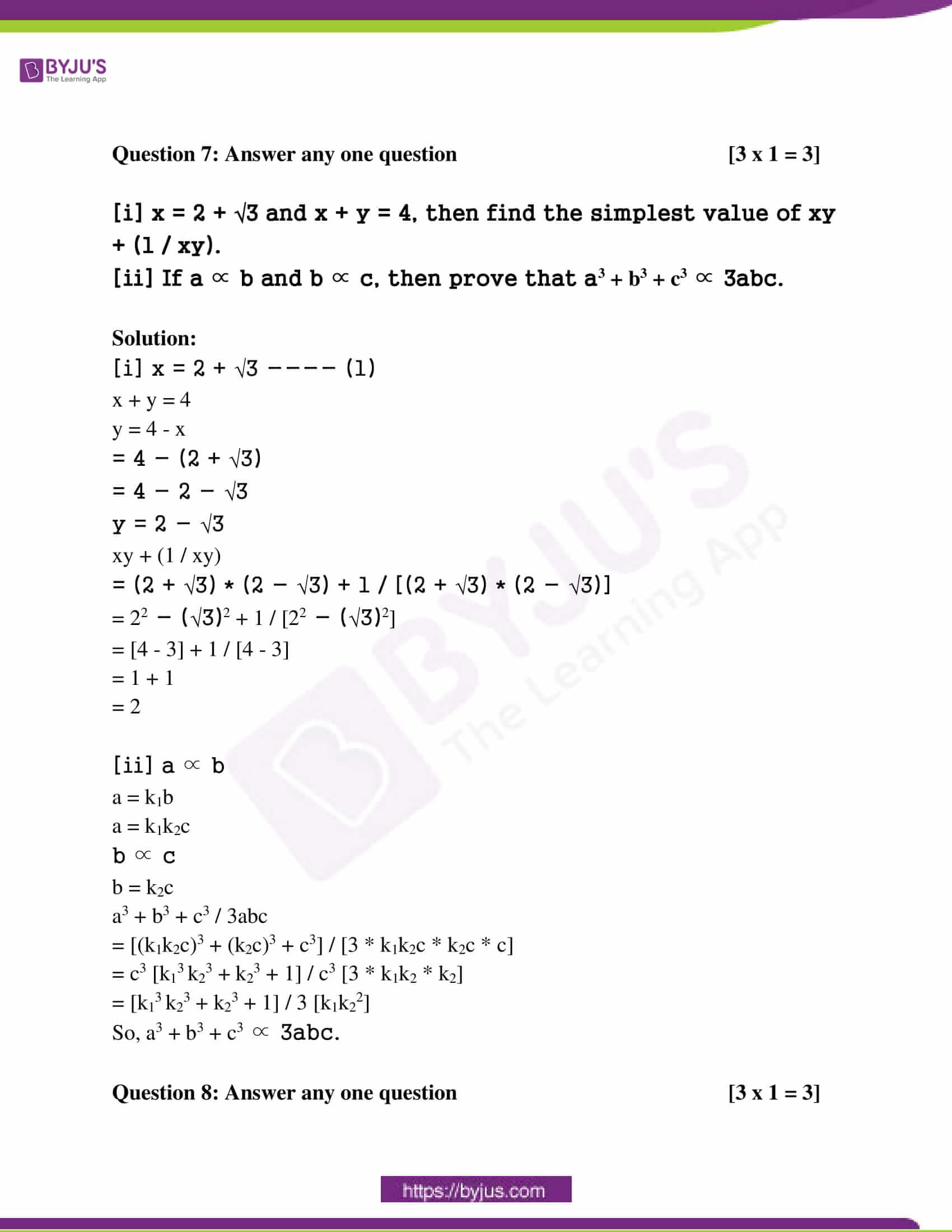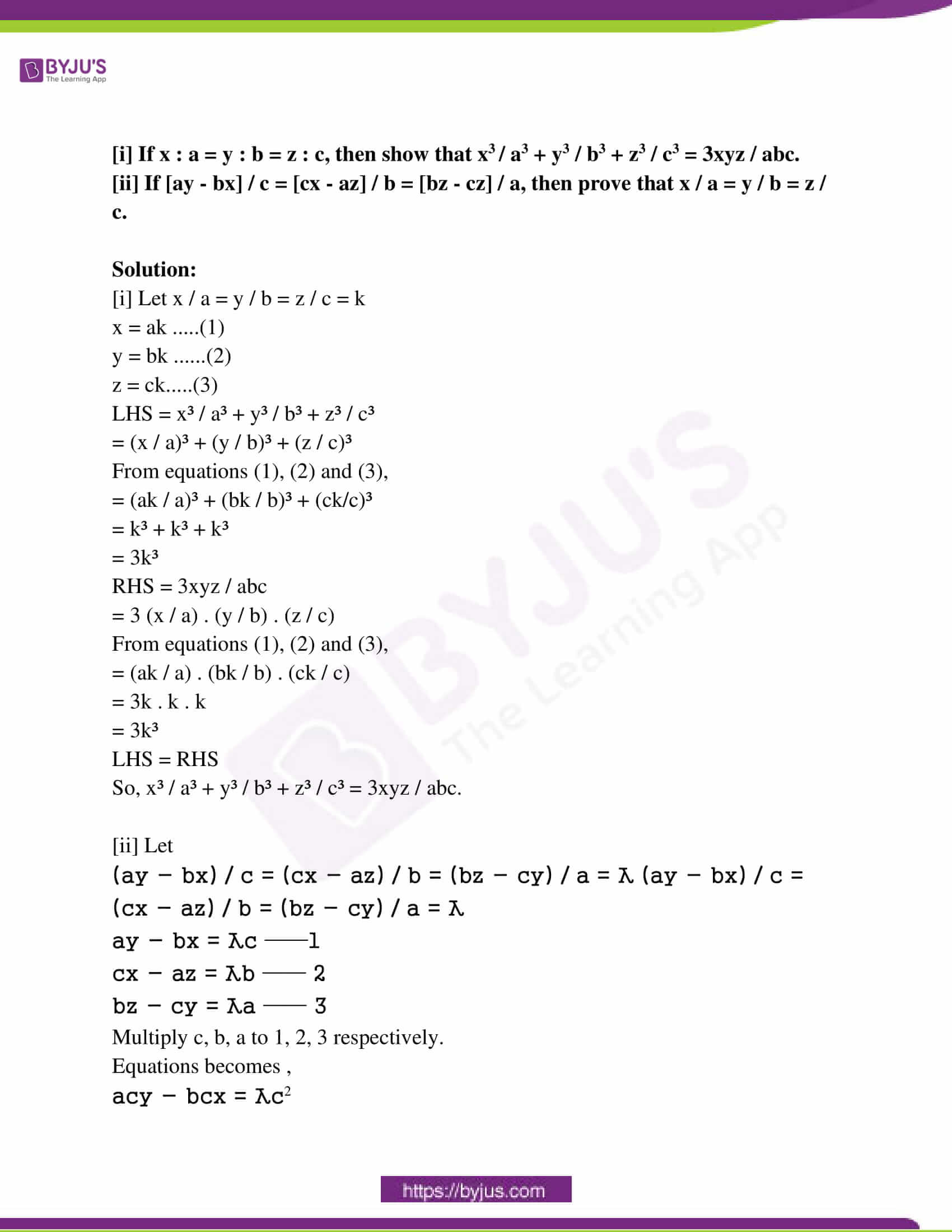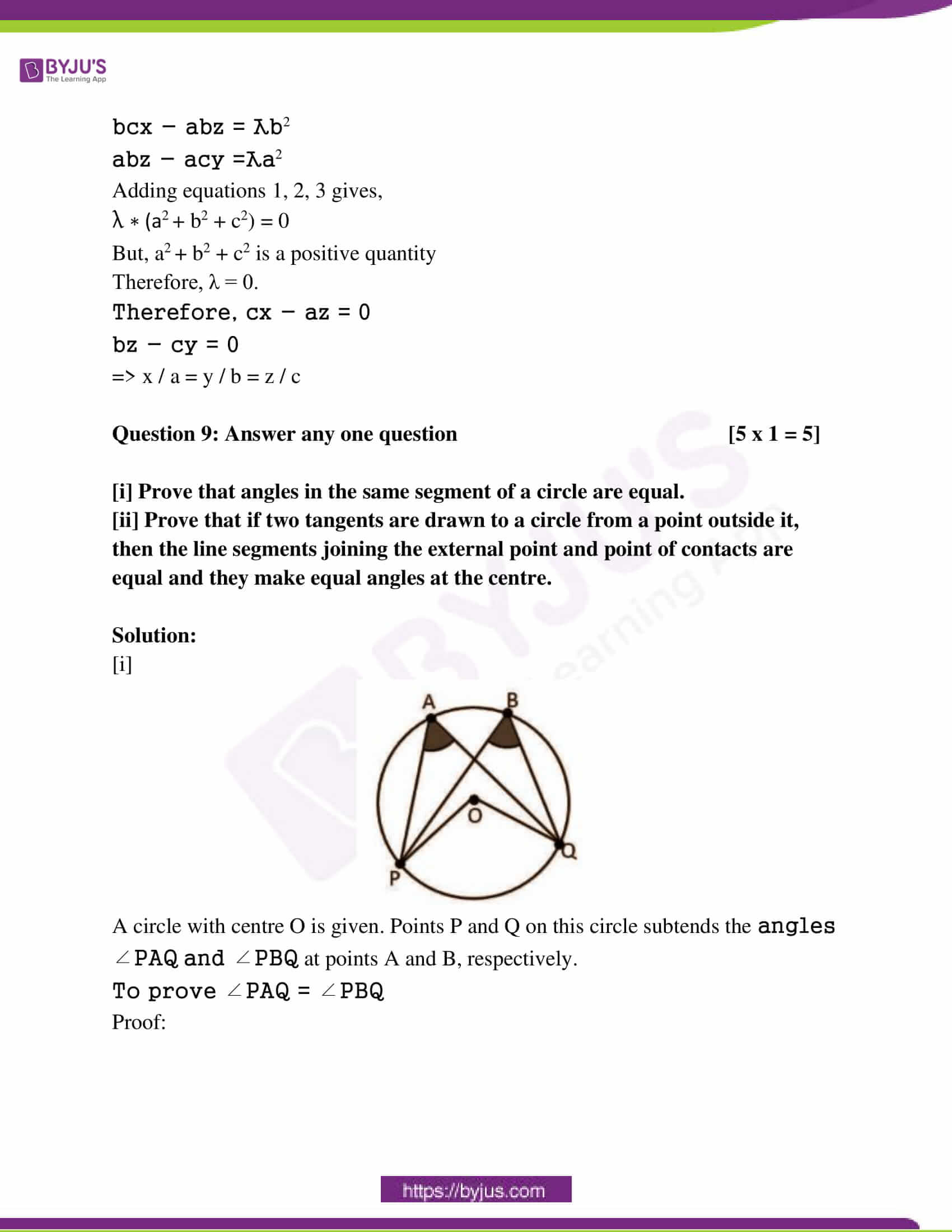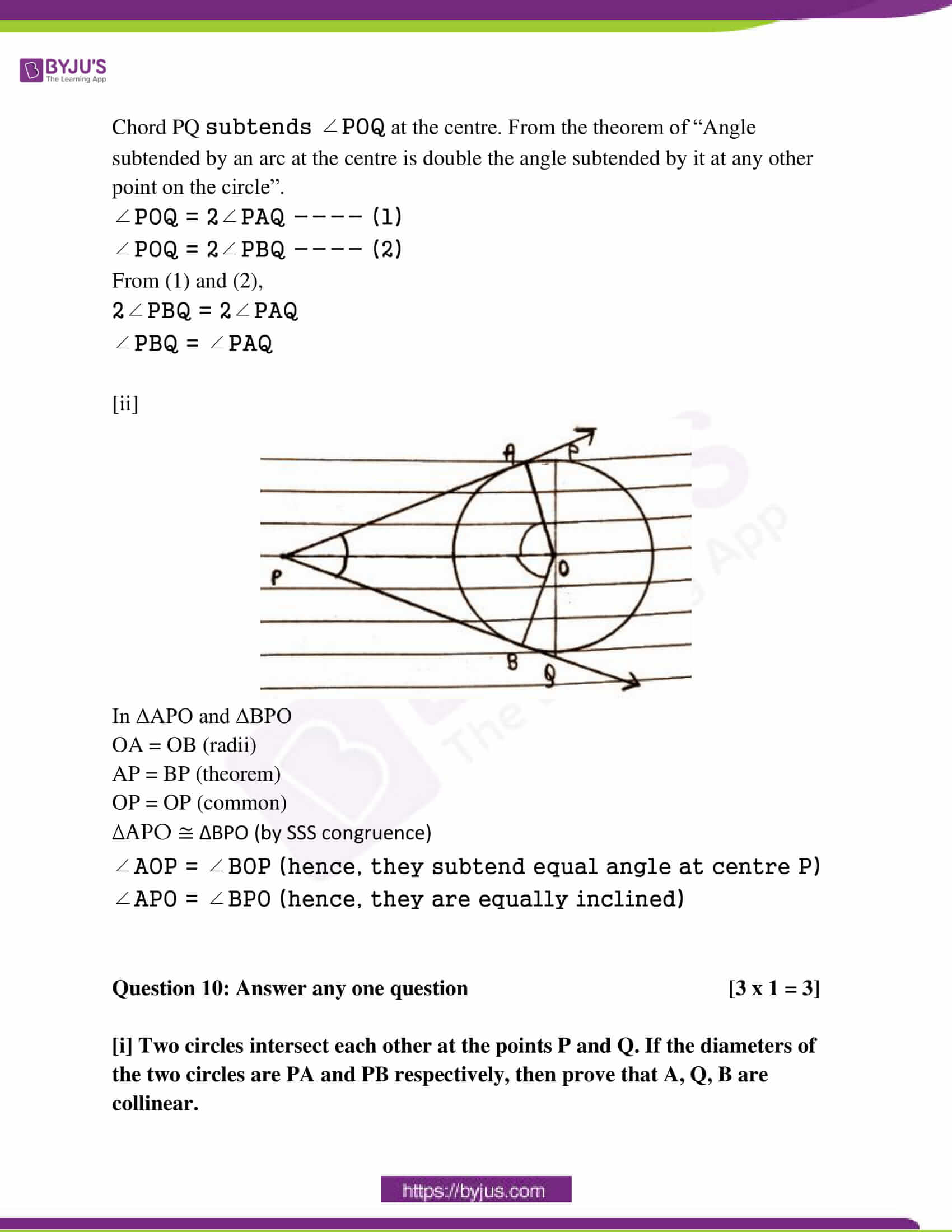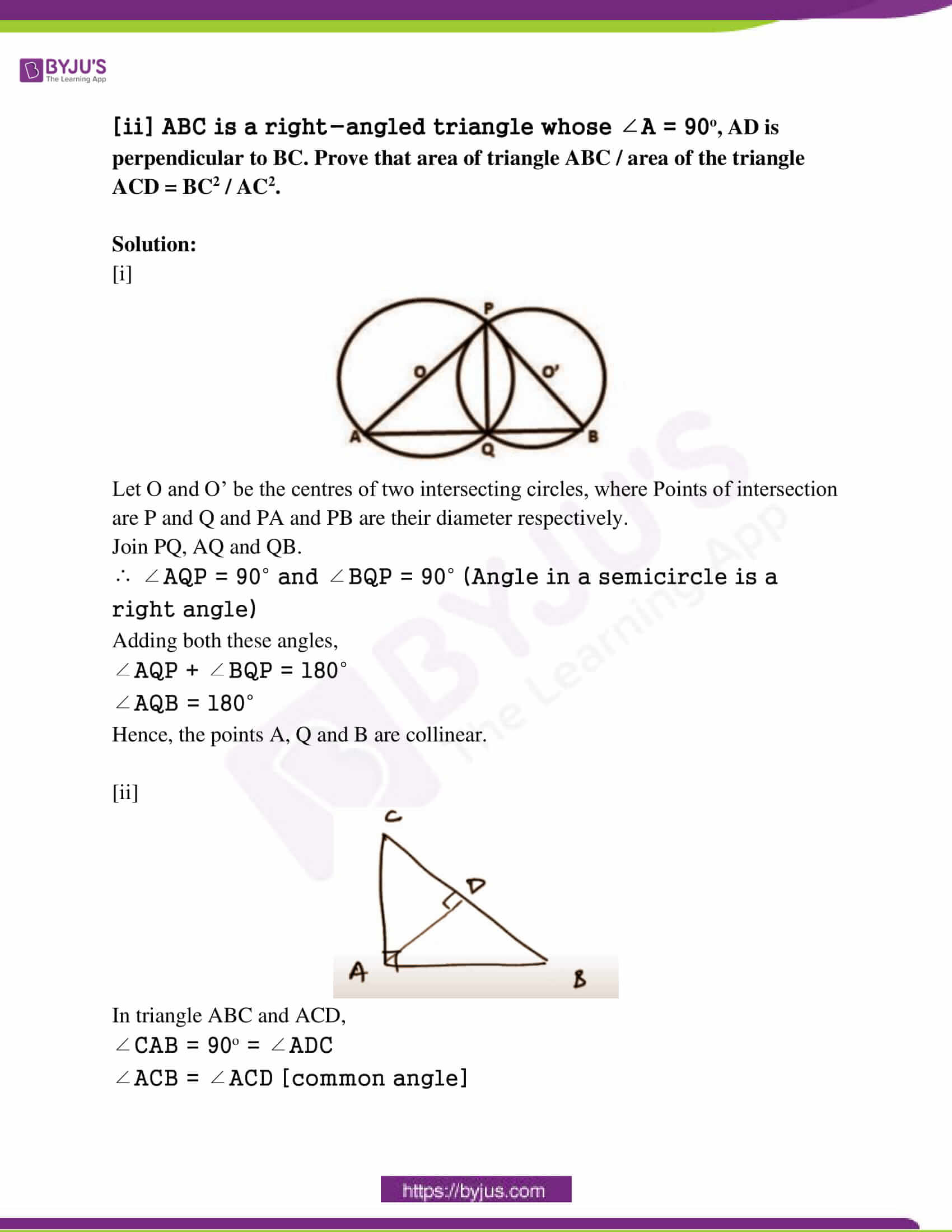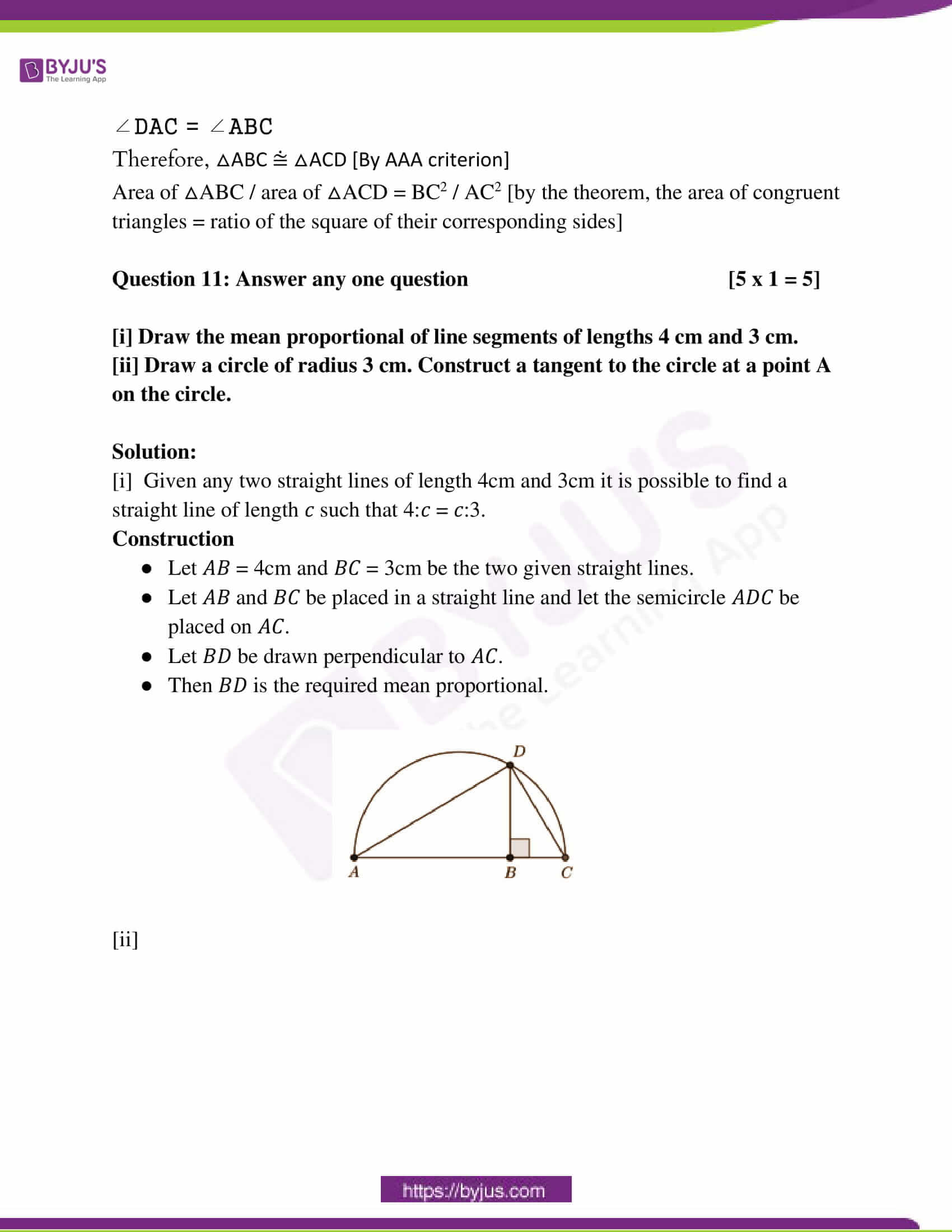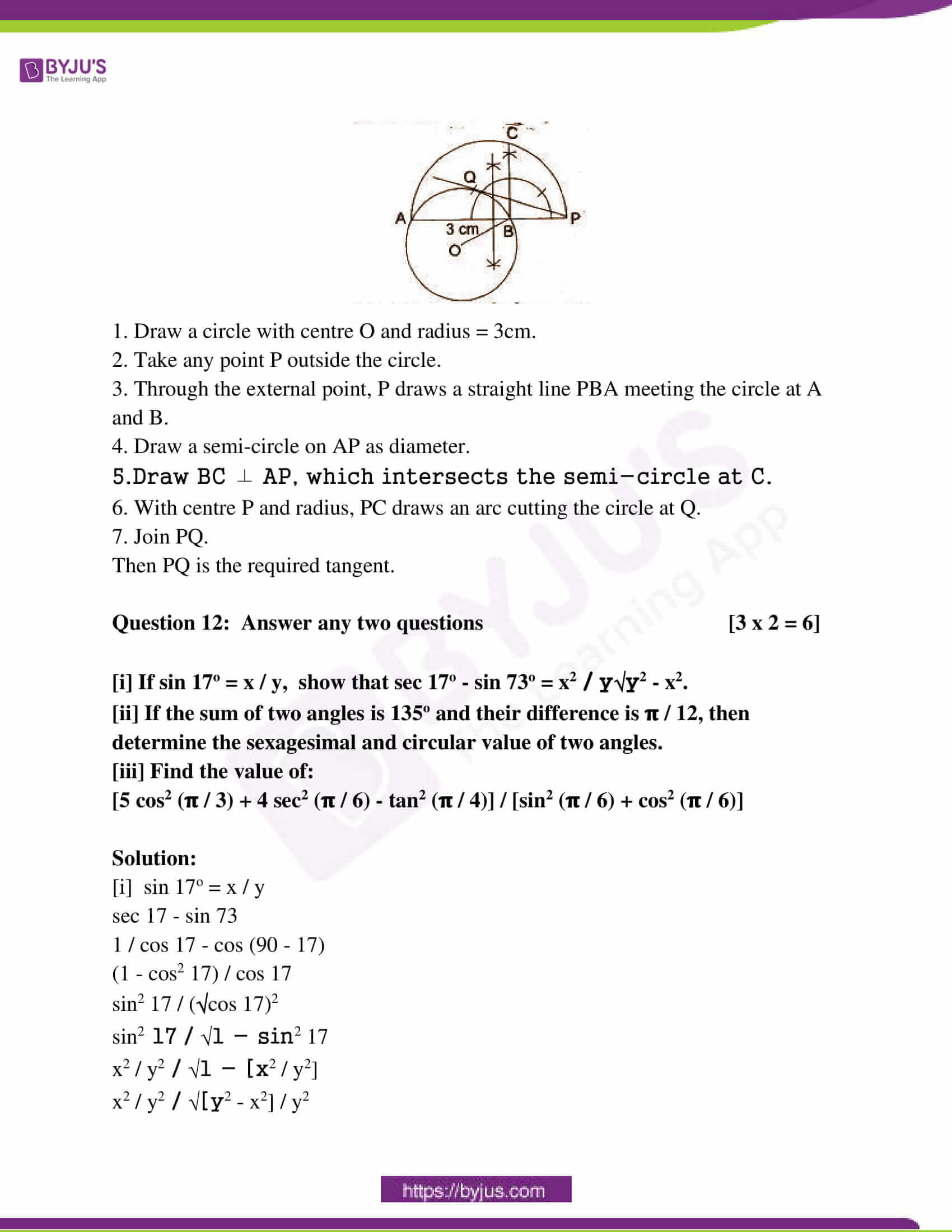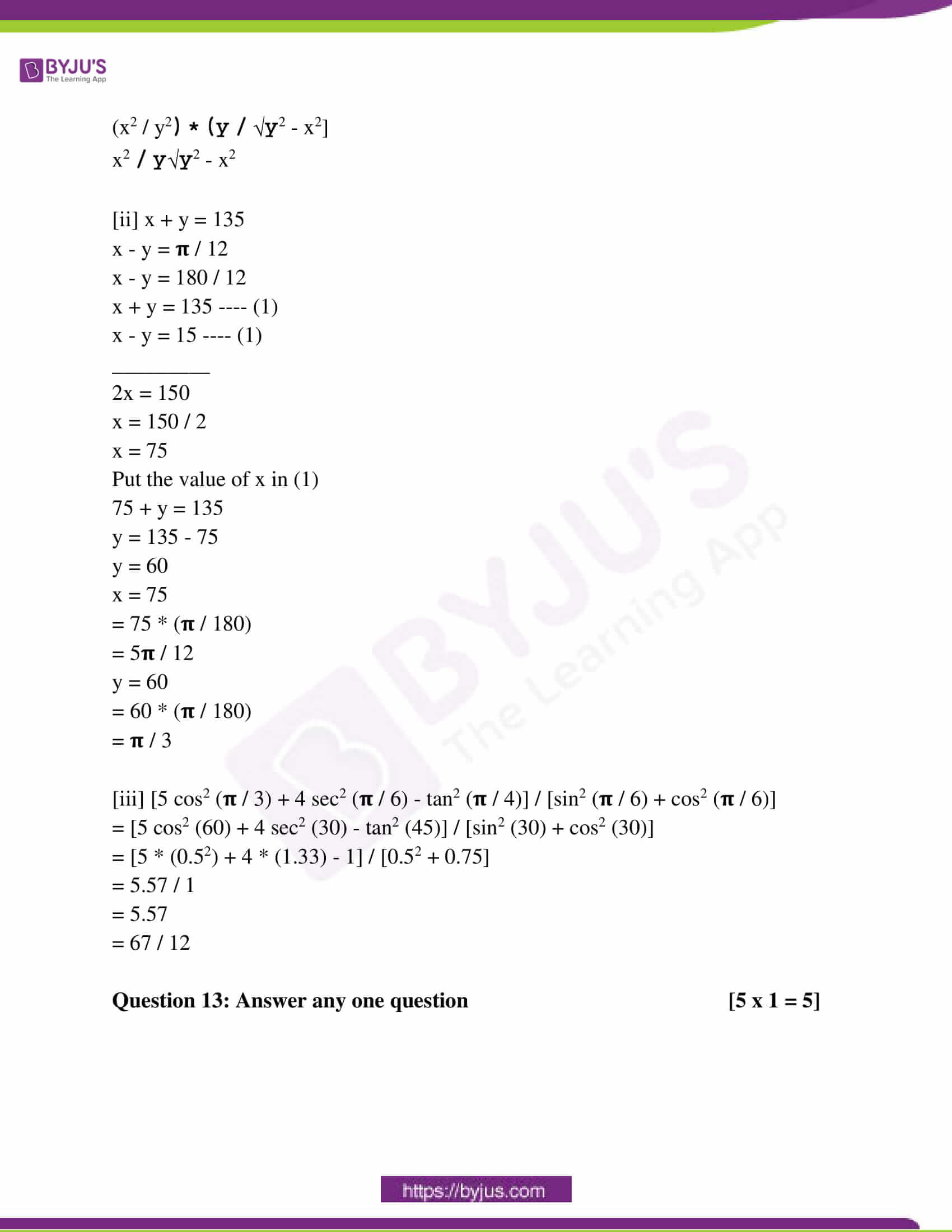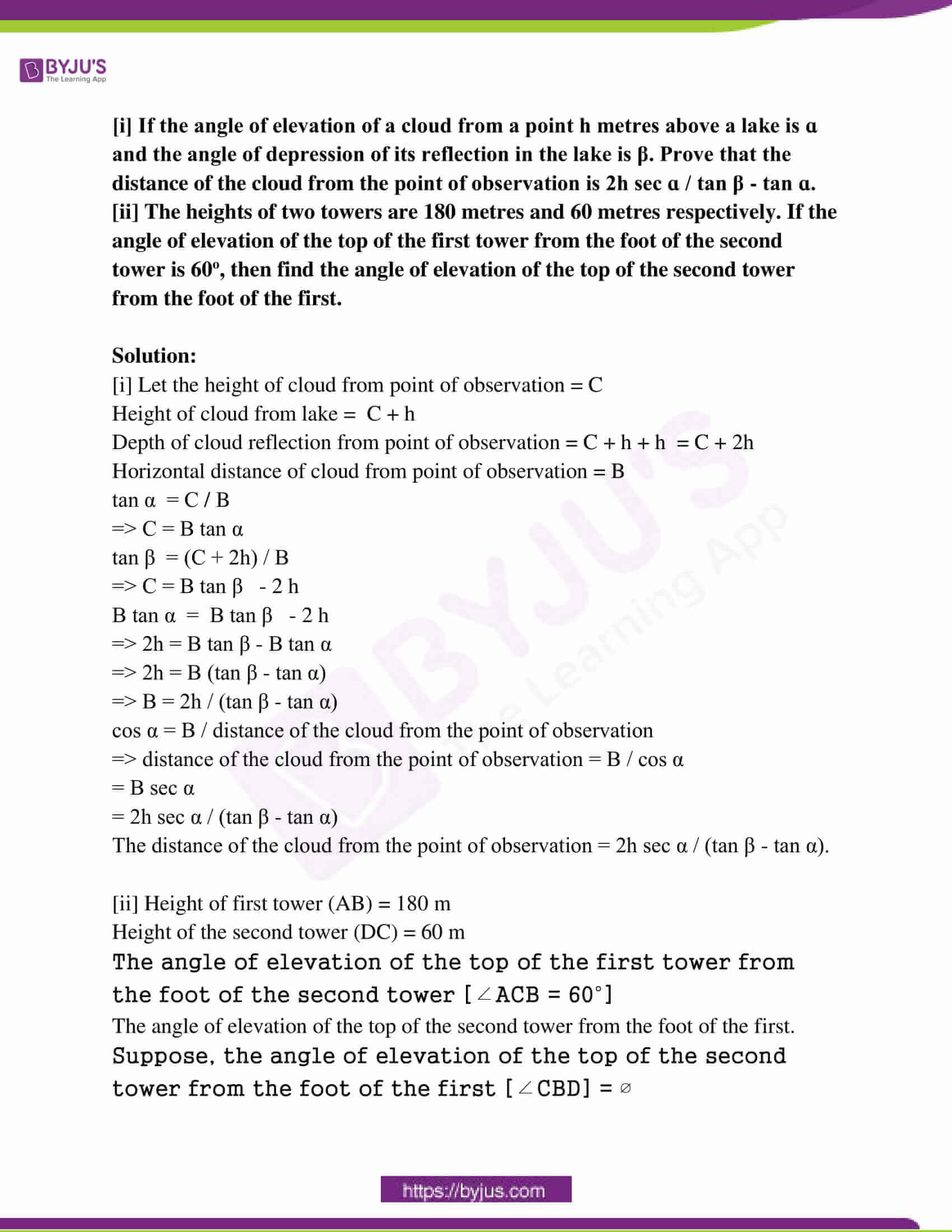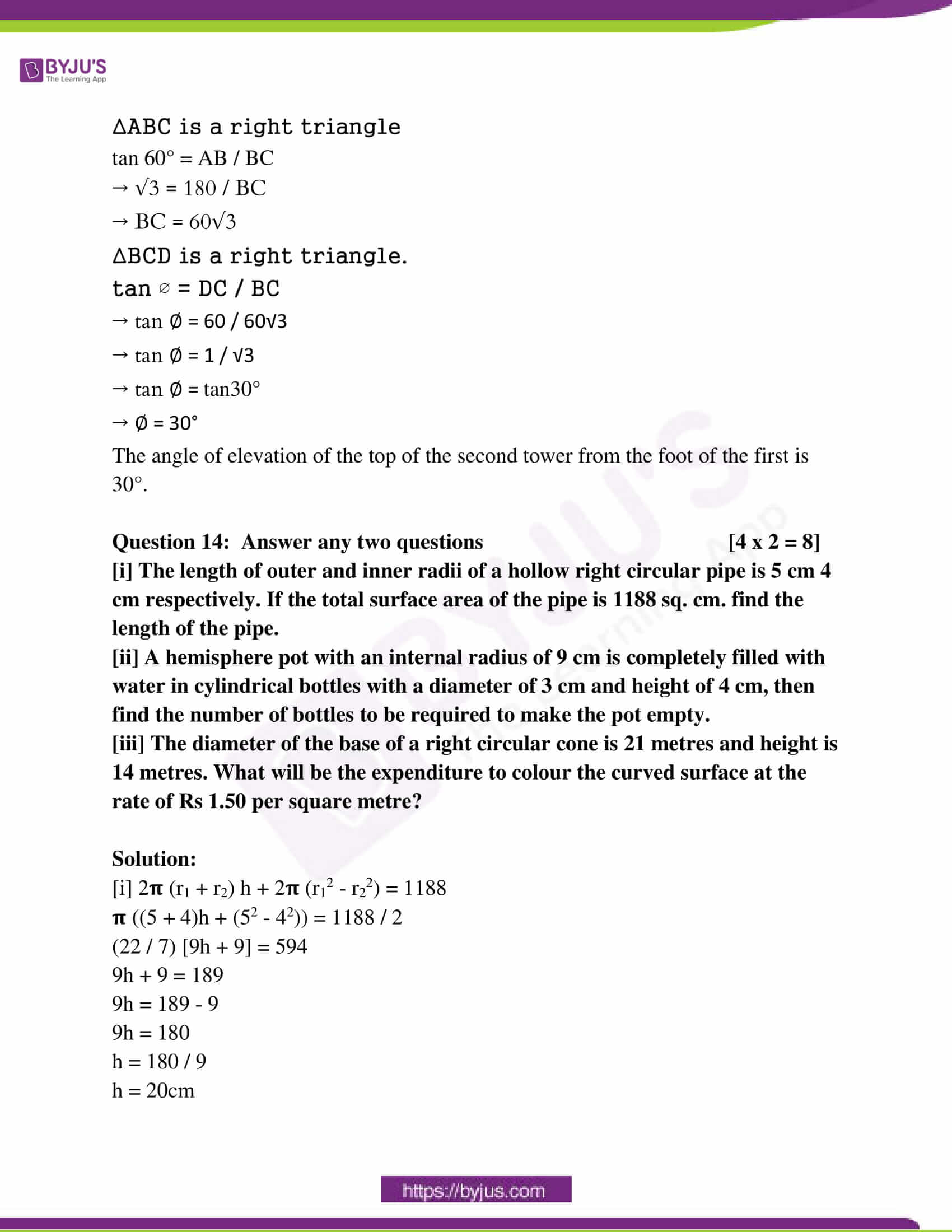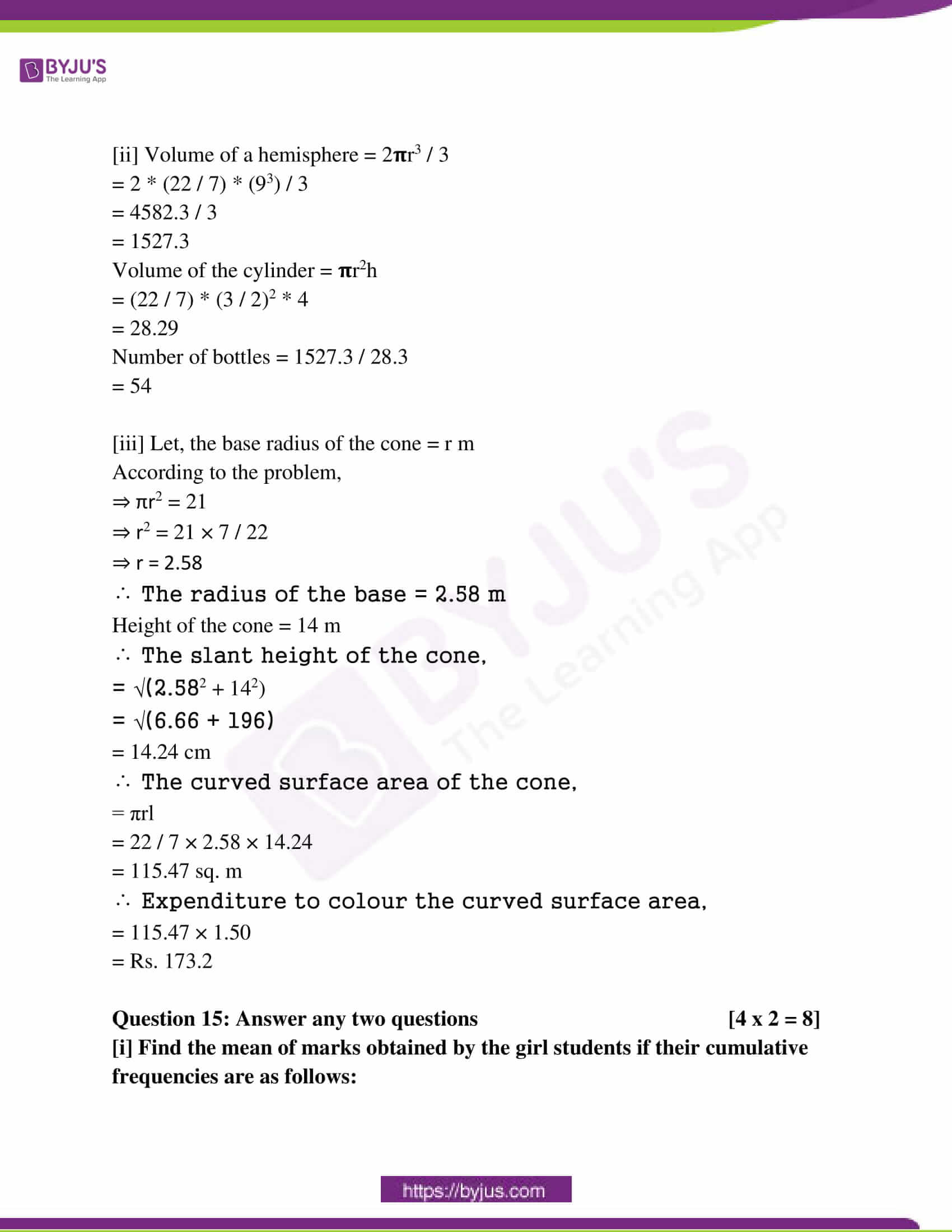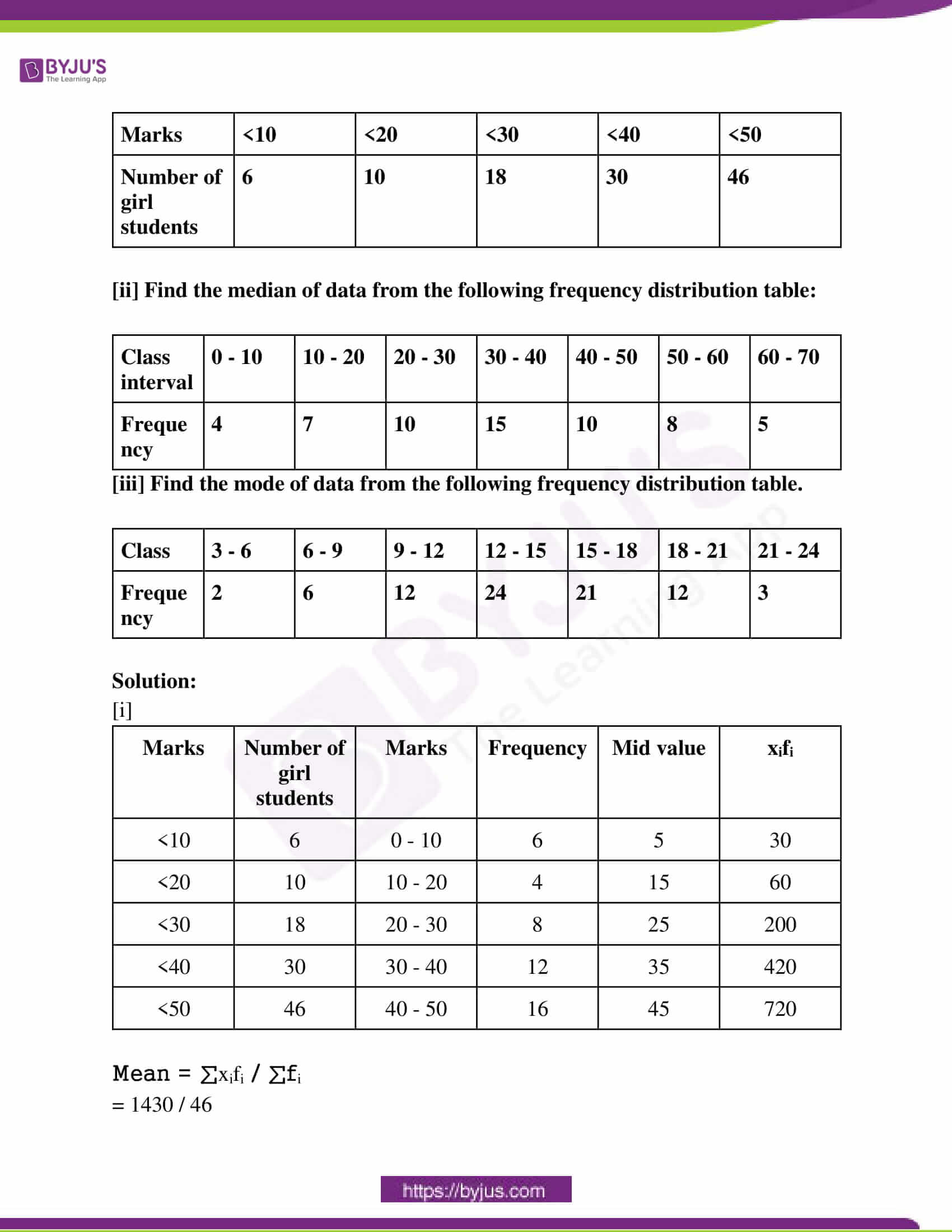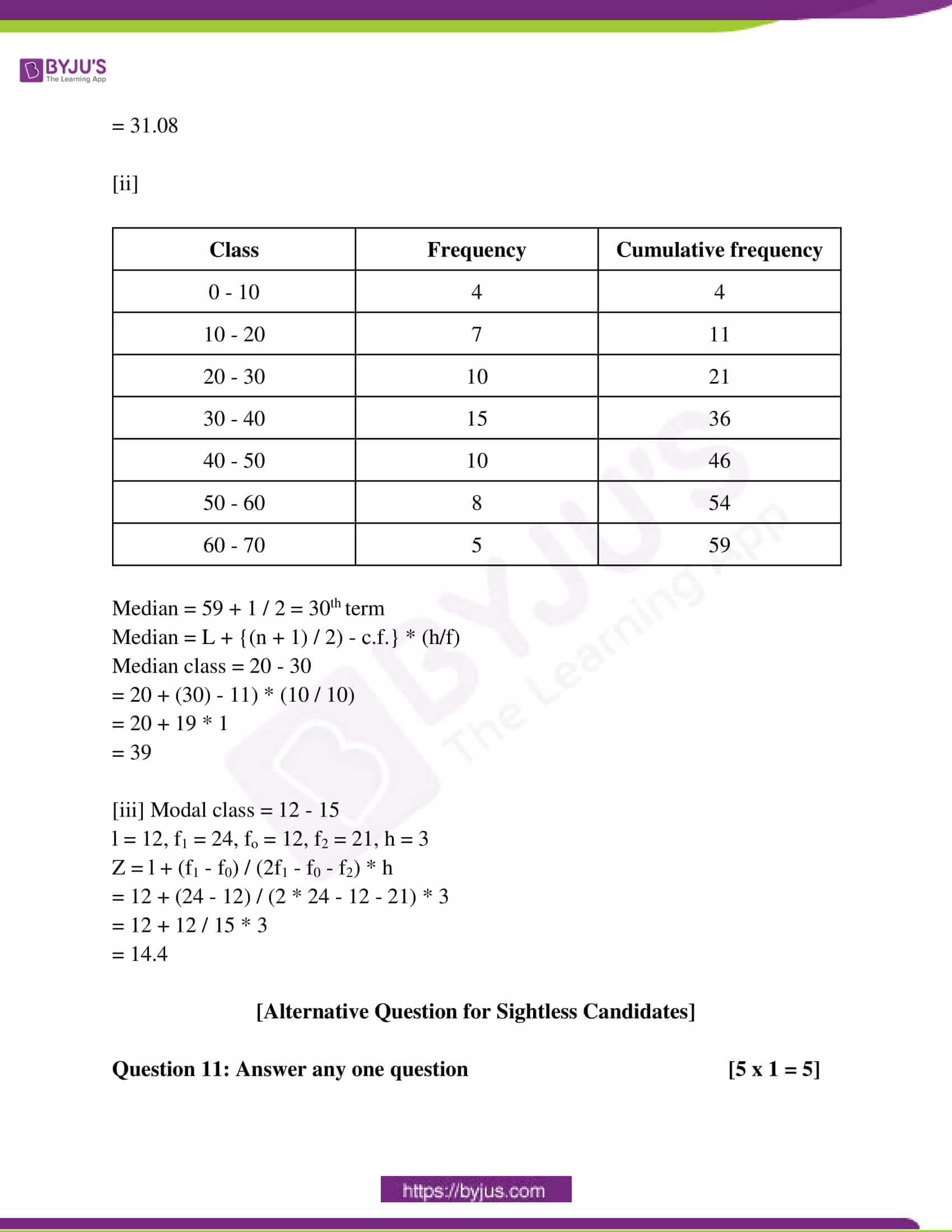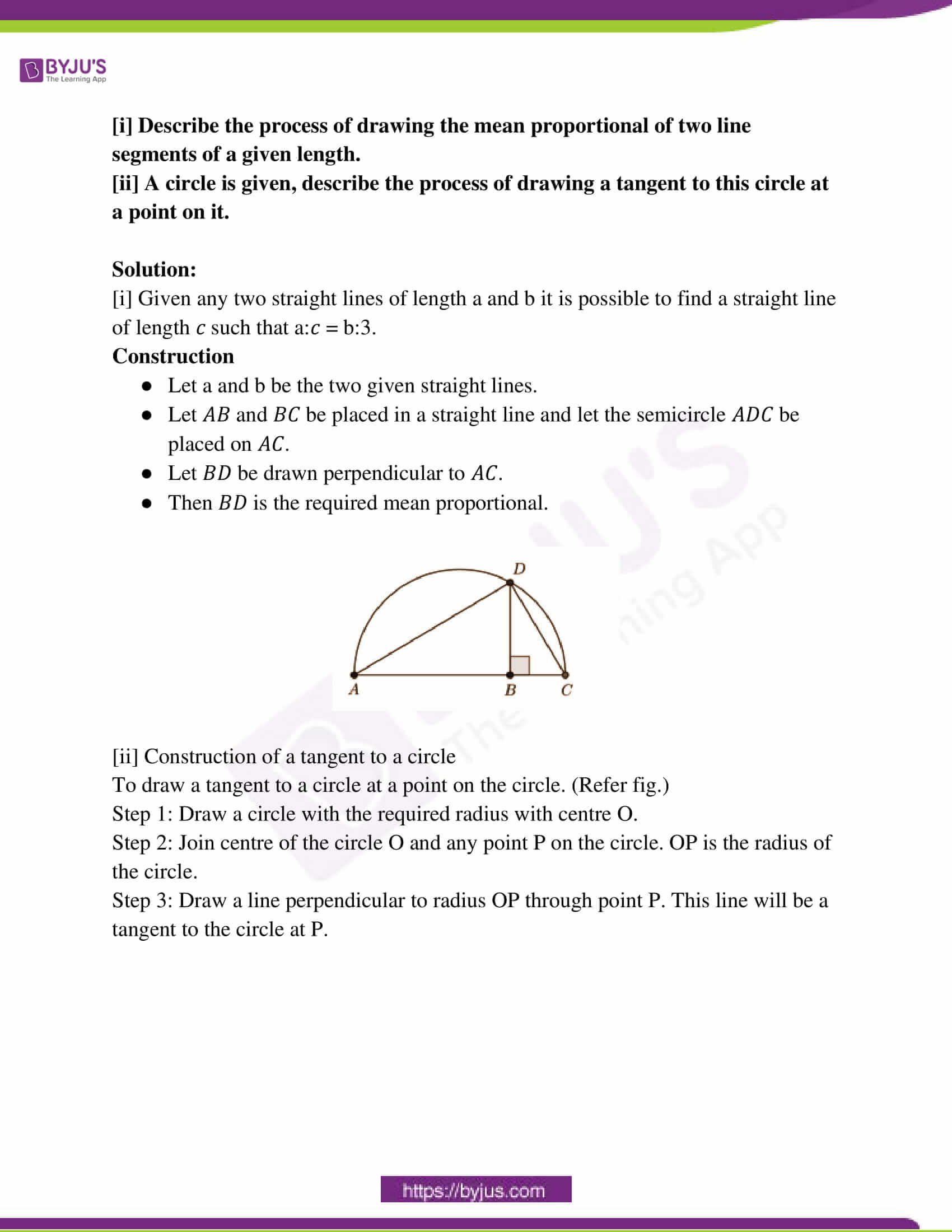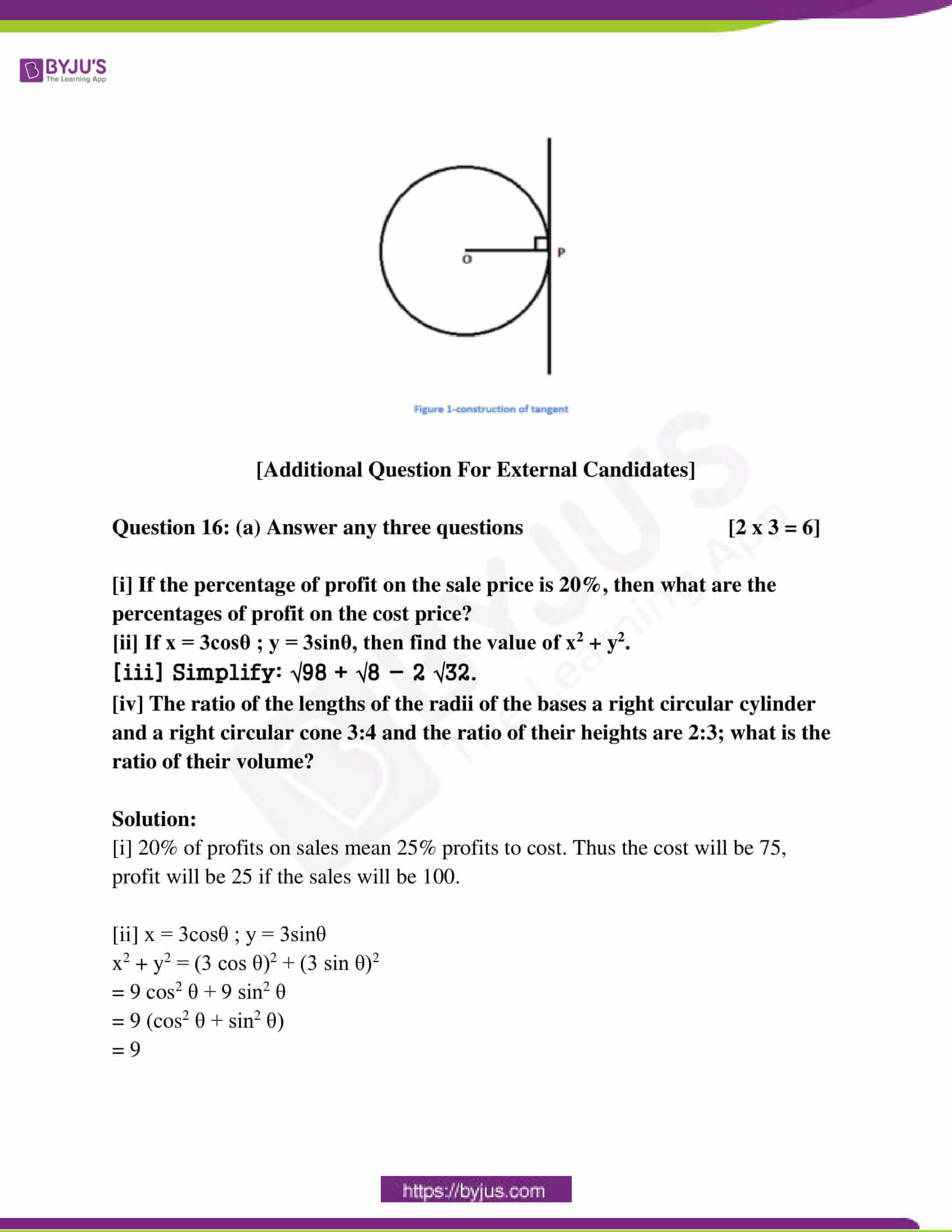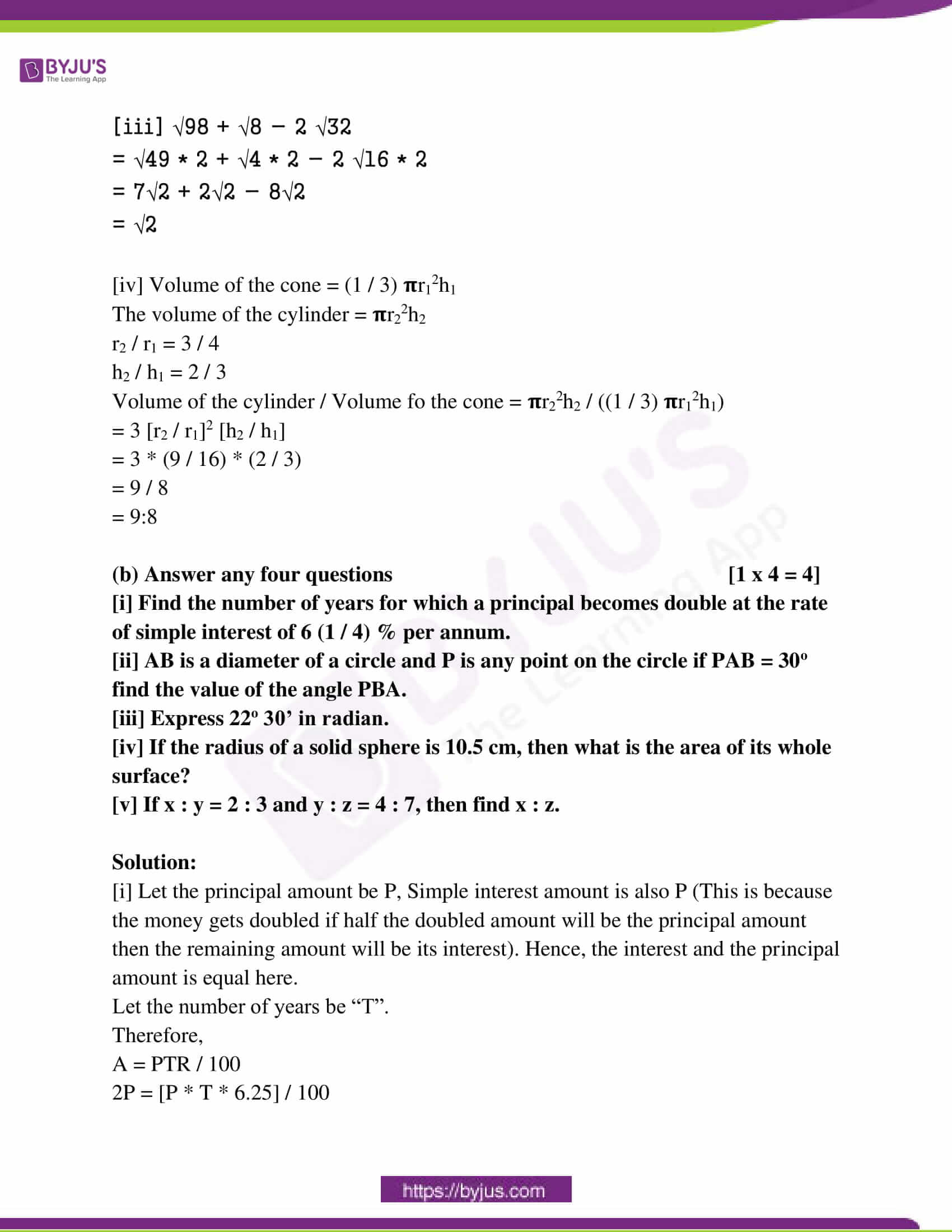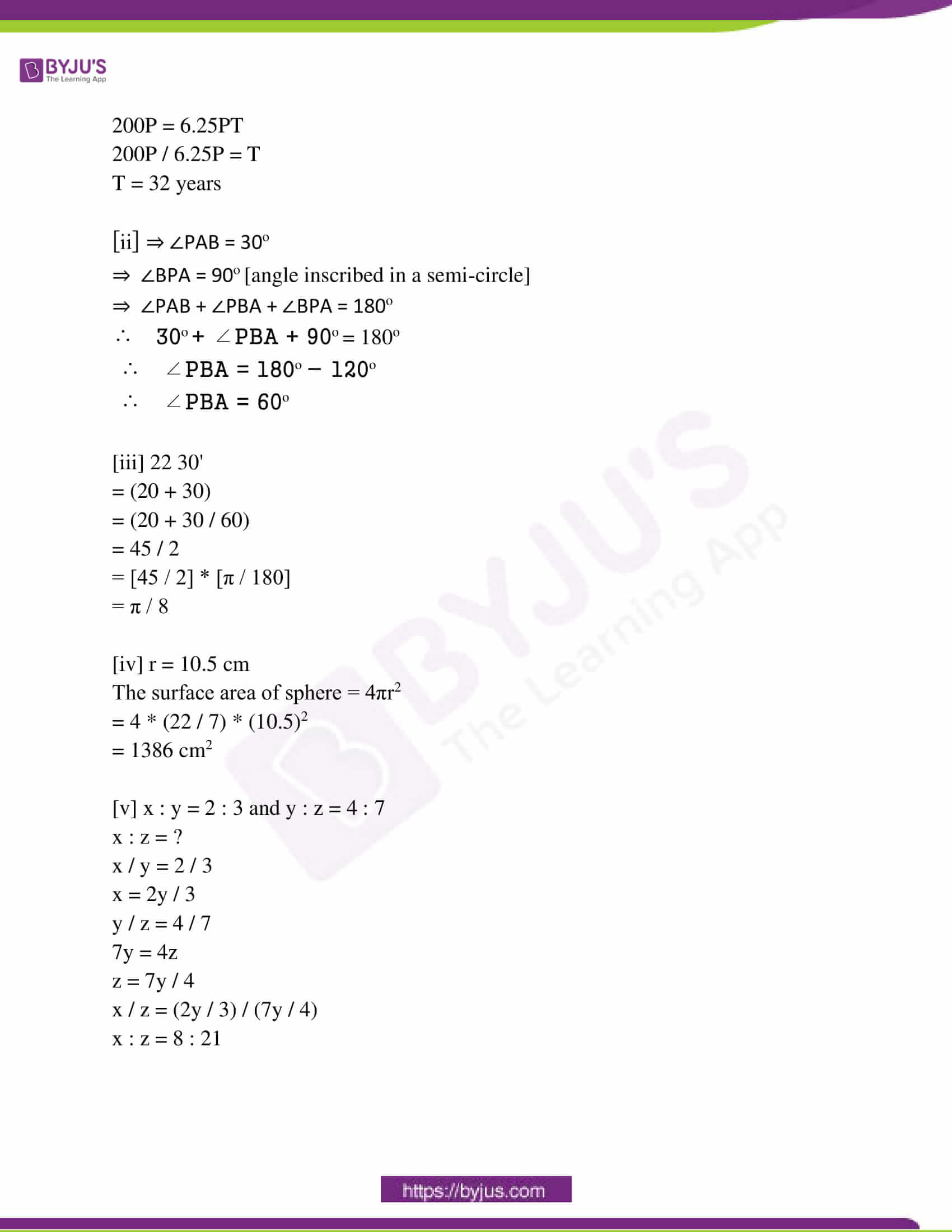Question 1: Choose the correct option in each case from the following questions : [1 x 6 = 6]

[i] If a principal becomes twice of it in 10 years, then the rate of simple

interest per annum is:

(a) 5% (b) 10% (c) 15% (d) 20%

Principal – x

Time – 10 year

Simple interest – 2x

According to the formula,

R= SI * 100 / 10 * 2x

R = 2x * 100 / 10 * x

R = 10%

[ii] The product of two roots of the equation x2 – 7x + 3 = 0 is:

(a) 7 (b) -7 (c) 3 (d) -3

x2 – 7x + 3 = 0

Roots are 7 + √(37) / 2 and 7 – √(37) / 2

Product = 72 – (√37)2 / 4 ( a + b × a – b = a2 – b2 )

= 49 – 37 / 4

= 12 / 4

= 3

[iii] The length of two chords AB and CD of a circle of centre O are equal

and AOB = 60o, then COD is:

(a) 30o (b) 60o (c) 120o (d) 180o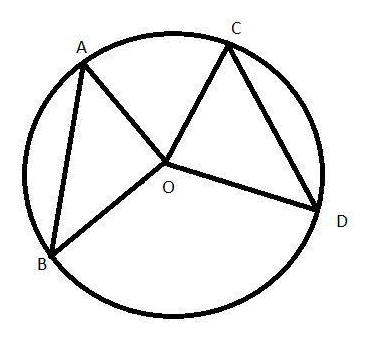Given: AB = CD and ∠AOB = 60°

To find: ∠COD

Consider ΔAOB and ΔCOD

AO = OC (Radius of the circle)

BO = OD (Radius of the circle)

AB = CD (Given)

Δ AOB ≅ ΔCOD (Side-Side-Side criteria)

∠COD = ∠AOB = 60° (Corresponding Parts of Congruent Triangles)

[iv] If the ratio of the volume of two right circular cones is 1:4 and the ratio

of radii of their bases is 4:5, then the ratio of their heights is:

(a) 1 : 5 (b) 5 : 4 (c) 25 : 16 (d) 25 : 64

v : V = 1 : 4

d : D = 4 : 5

a : A = (42) : (52) = 16 : 25.

h : H = ?

v = (1 / 3) * a * h and V = (1 / 3) * A * H

(1 / 4) = (16 / 25) * (h / H)

(h / H) = (1 / 4) * (25 / 16) = (25 / 64)

h : H = 25 : 64

[v] If sin θ – cos θ = 0, (0o < θ < 90o) and sec θ + cosec θ = x, then the value of x

is:

(a) 1 (b) 2 (c) √2 (d) 2√2

Let us assume θ to be “p”.

sin p – cos p = 0

=> (sin p – cos p)² = 0²

=> sin²p + cos²p = 2 sin p cos p

=> 1 / 2 = sin p cos p

Also, (sin p + cos p)²

= sin²p + cos²p + 2 sin p cos p

= 1 + 2 (1 / 2)

= 1 + 1

= 2

Therefore, (sin p + cos p) = √2

Now, sec p + cosec p = x

=> 1 / cos p + 1 / sin p = x

=> (sin p + cos p) / sin p cos p = x

=> 2√2 = x

[vi] The mode of 1, 3, 2, 8, 10, 8, 3, 2, 8, 8 is:

(a) 2 (b) 3 (c) 8 (d) 10

Question 2: Fill up the blanks (any five) [1 x 5 = 5]

[i] Anisur invests Rs 500 for 9 months in a business and Devid invests Rs

600 for 5 months in the same business, the ratio of their profits will be ________. [Answer: 3:2]

The amount each will have earned at the end of the investment periods is given by:

Anisur: 500 × 9 = 4500

Devid: 600 × 5 = 3000

The ratio of their profits is therefore given by:

Anisur:Devid

4500 / 3000 = 3 / 2

The ratio of their profits is 3:2.

So, if they were to share the profits, they would do so in the ratio 3:2.

[ii] The roots of the quadratic equation ax2 + 2bx + c = 0 (a ≠ 0) are real and unequal, then b2 = ________. [Answer: 0]

[iii] If the sum of two angles is ________, then they are called supplementary angles. [Answer: 180o] [iv] Maximum value of sin 3θ is ________. [Answer: 1]

[v] One solid sphere is melted and a solid right circular cylinder is made,

then ________ of the sphere and the cylinder will be equal. [Answer: volume]

[vi] Ages of some students are (in years) 10, 11, 9, 7, 13, 8, 14; the median of

the ages of those students is ________ years. 

Question 3: Write True or False (any five) [1 x 5 = 5]

[i] The amount of Rs 2p in t years at the rate of simple interest of (r / 2) % per annum is Rs. [2p + (prt / 100)]. [Answer: True]

[ii] If 2a = 3b = 4c then a : b : c = 2 : 3 : 4. [Answer: False]

[iii] If the ratio of the lengths of three sides of a triangle is 5:12:13, then the triangle will always be a right-angled triangle. [Answer: True]

[iv] The angle formed by rotating a ray about its endpoint in anticlockwise

[v] If n is an even number, then median is the mean of (n / 2)th and {(n / 2) – 1}th observation. [Answer: False]

[vi] If the length of the radius of the base of a right circular cone be halved

and its height is doubled, then the volume remains the same. [Answer: False]

Question 4: Answer any ten questions [2 x 10 = 20]

(i) If the ratio of a principal and the amounts for 5 years is 5:6, then find

the rate of simple interest per annum.

Solution:

Let the principal be 5P and the amount is 6P.

SI = 6P – 5P = P

r = 100 * I / P * t

= 100 * P / 5P * 5

= 4%

(ii) In a business, A and B get Rs 1,050 as profit. If the principal and profit

of A be Rs 900 and Rs 630, respectively. Find the principal of B.

Solution:

Profit of A + B = 1050

Profit of A = 630

Profit of B = 1050 – 630 = 420

Let the principal of B be x.

Principal of A / Principal of B = Profit of A / Profit of B

900 / x = 630 / 420

900 / x = 1.5

900 / 1.5 = x

x = Rs. 600

(iii) If x ∝ y, y ∝ z and z ∝ x, find the product of three variation constants.

Solution:

x ∝ y, y ∝ z and z ∝ x

It can be written as,

x = ky, y = mz and z = nx where k, m and n are constants of variations.

Put the value of y in x,

x = kmz

Now put the value of z in x,

⇒ x = kmnx

⇒ kmn = 1

(iv) If the roots of the quadratic equation 5x2 – 2x + 3 = 0 be ɑ and β, find the value of [1 / ɑ] + [1 / β].

Solution:

5x2 – 2x + 3 = 0

a = 5, b = -2, c = 3

ɑ + β = – b / a

= 2 / 5

ɑ * β = c / a

= 3 / 5

[1 / ɑ] + [1 / β]

= ɑ + β / ɑ * β

= (2 / 5) / (3 / 5)

= 2 / 3

(v) The point O is situated within the rectangular region ABCD in such a way that OB = 6 cm, OD = 8 cm and OA = 5 cm. Determine the length of OC.

Solution: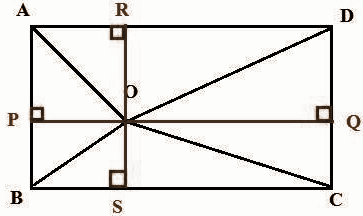O is any point inside a rectangle ABCD such that OB = 6 cm, OA = 5 cm and OD = 8 cm.

In ΔAPO, Using Pythagoras theorem,

OA2 = AP2 + OP2

In ΔCSO, Using Pythagoras theorem

OC2 = CS2 + OS2

OA2 + OC2 = AP2 + OP2 + CS2 + OS2

OA2 + OC2 = OD2 + OB2

Substitute OB = 6 cm, OA = 5 cm and OD = 8 cm,

52 + OC2 = 62 + 82

25 + OC2 = 36 + 64

OC2 = 100 – 25

OC2 = 75

OC = 5√3 cm

(vi) In a right-angled triangle ABC, ∠ABC = 90o, AB = 3 cm, BC = 4 cm and the perpendicular BD on the side AC from the point B which meets the side AC at the point D. Determine the length of BD.

Solution:

AB = 3cm

BC = 4cm

AC = √42 + 32

= √16 + 9

= √25

AC = 5cm

[1 / 2] * BC * AB = [1 / 2] * AC * BD

[1 / 2] * 4 * 3 = [1 / 2] * 5 * BD

12 =  BD

12 / 5 = BD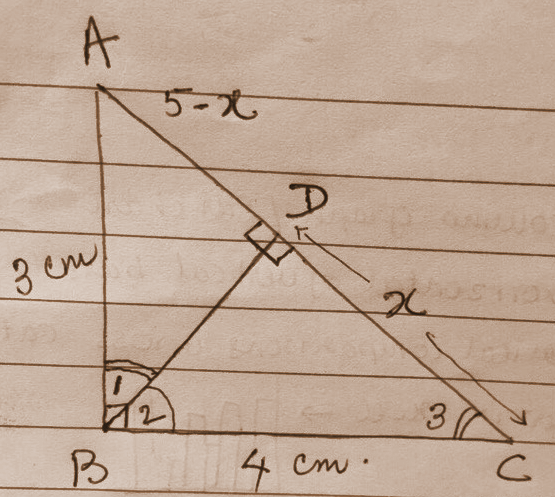(vii) The lengths of radii of two circles are 8 cm and 3 cm and the distance between two centres is 13 cm. What is the length of the direct common tangent of two circles?

Solution: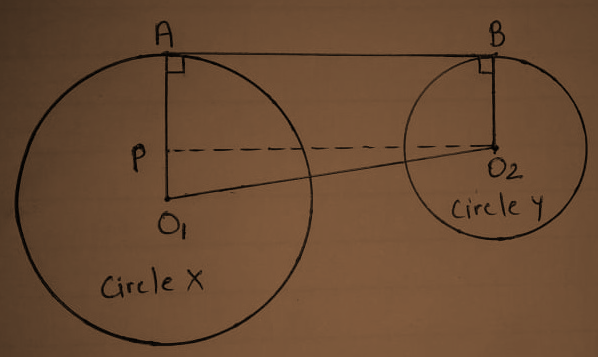Let O1 and O2 be the centres of circles X and circle Y, respectively.

⇒ The radius of circle X is O1A = 8cm.

⇒ The radius of circle Y is O2B = 3cm.

⇒ Distance between the centres of the two circles O1O2 = 13cm.

⇒ To find the length of a common tangent AB, construct a line segment PO2, which is parallel to AB.

⇒ PO1 = O1A − O2B

= 8 − 3

= 5cm

⇒ △PO1O2 is a right-angled triangle.

∴ PO2 = (O1O2)2 − (O1P)2 [By using Pythagoras theorem]

∴ PO2= √132 − 52

= √169 − 25

​= √144

∴ PO2 = 12cm

⇒ Hence, the length of common tangent AB = PO2 = 12cm.

(viii) What is the circular measure of an angle formed by the rotation of the hour hand of a clock in one-hour duration?

Solution:

The hour hand of a clock rotates in one day is about 360°.

Number of hours in a day (or night) = 12

Number of hours in a day / degree covered in a day (or night) is,

= 360o / 12

= 30°

(ix) If tan 4θ tan 6θ = 1 and 6θ is a positive acute angle, find the value of θ.

Solution:

tan 4θ tan 6θ = 1

tan 4θ = 1 / tan 6θ = cot 6θ

tan 4θ = tan (𝛑 / 2 – 6θ)

4θ = (𝛑 / 2 – 6θ)

4θ + 6θ = (𝛑 / 2)

10θ = 90

θ = 90 / 10

θ = 9o

(x) The height of a right circular cone is 12 cm and its volume is 100 𝛑cm3. Find the lateral height of the cone.

Solution:

Since volume of cone = [1 / 3] Πr²h

h = 12cm

Volume = 100Πcm³

[1 / 3] x Π x r² x 12 = 100Π

4Π r² = 100Π

r2 = 100 / 4

r2 = 25

r = 5cm

Slant height,

l² = 5² + 12²

l² = 169

l = 13cm

(xi) Curved surface areas of two spheres are in a ratio 1:4. Find the ratio of their volumes.

Solution:

Let the radius of the two spheres are ‘r’ and ‘R’.

The ratio of the surface area of two spheres = 1: 4

⇒ 4πr2 : 4πR2 = 1 : 4

⇒ r : R = 1 : 2

The ratio of volumes of two spheres is

= (4 / 3) πr3 : (4 / 3) πR3

= r3 : R3

= 13 : 23

= 1: 8

(xii) If ui = xi – 35 / 10, ∑fiui = 30 and ∑fi = 60, then determine the value of mean.

Solution:

ui = xi – 35 / 10 —- (1)

∑fiui = 30 and ∑fi = 60

ui = xi – a / h —- (2)

From (1) and (2),

a = 35, h = 10

Mean = a + [∑fiui / ∑fi] * h

= 35 + [30 / 60] * 10

= 35 + [0.5] * 10

= 35 + 5

= 40

Question 5: Answer any one question [5 x 1 = 5]

(i) The price of a machine in a factory of your uncle depreciates at the rate of 10% every year. If its present price is Rs 6,000 then what will be its price after 3 years?

Solution:

The price after 3 years = P(1 – (r / 100))n

= 6000 * [1 – (10 / 100)]3

= 6000 * [90 / 100]3

= 6000 * 0.729

= Rs. 4374

(ii) Three friends invested Rs 1,20,000 Rs 1,50,000 and Rs, 1,10,000, respectively to purchase a bus. The first person is a driver and the other two are conductors. They decided to divide 2 / 5th of the profit among themselves in the ratio of 3:2:2 according to their work and remaining in the ratio of their capitals. If they earn Rs 29,260 in one month, find the share of each of them.

Solution:

The ratio of investments of three friends

= 120000:150000:110000

= 12:15:11

Profit earned in one month = ₹ 29260

[2 / 5]th of profit ₹ 29260

= (2 / 5) * 29260

= ₹ 11704

From the profit of ₹11704,

1st friend gets (11704 × 3) / 7 = ₹ 5016

2nd friend gets (11704 × 2) / 7 = ₹ 3344

3rd friend gets (11704 × 2) / 7 = ₹ 3344

The remaining money from the actual profit made

= ₹ (29260 – 11704)

= ₹ 17556

Now, from ₹ 17556,

1st friend gets (17556 × 12) / 38 = ₹ 5544

2nd friend gets (17556 × 15) / 38 = ₹ 6930

3rd friend gets (17556 × 11) / 38 = ₹ 5082

Now, the first, second and third friends obtain a total amount of ₹10560, ₹10274, ₹8426.

Question 6: Answer any one question [3 x 1 = 3]

(i) Solve (1 / [x – 3]) + (1 / [x + 5]) = 1 / 6.

(ii) The product of two consecutive positive odd numbers is 143. Construct the equation and determine the numbers by applying Sridhara Acharyaa’s formula.

Solution:

(i) (1 / [x – 3]) + (1 / [x + 5]) = 1 / 6

By taking LCM,

(x + 5) – (x – 3) / (x – 3) (x + 5) = 1 / 6

(x + 5) – (x + 3) / x2 + 5x – 3x – 15 = 1 / 6

8 / x2 + 2x – 15 = 1 / 6

x2 + 2x – 15 = 48

=> x2 + 2x – 15 – 48 = 0

=> x2 + 2x – 63 = 0

=> x2 + 9x – 7x – 63 = 0

=> x (x + 9) – 7 (x + 9) = 0

=> (x + 9) (x – 7) = 0

=> x + 9 = 0

=> x = -9

x – 7 = 0

=> x = 7

So, x = 7 or -9.

(ii) Let the two numbers be x and x + 2.

x ( x + 2) = 143

x2 + 2x – 143 = 0

x2 + 13x − 11x − 143 = 0

x (x + 13) − 11 (x + 13) = 0

(x − 11) (x + 13) = 0

x = 11,−13

The numbers are 11 and 13.

Question 7: Answer any one question [3 x 1 = 3]

[i] x = 2 + √3 and x + y = 4, then find the simplest value of xy + (1 / xy).

[ii] If a ∝ b and b ∝ c, then prove that a3 + b3 + c3 ∝ 3abc.

Solution:

[i] x = 2 + √3 —- (1)

x + y = 4

y = 4 – x

= 4 – (2 + √3)

= 4 – 2 – √3

y = 2 – √3

xy + (1 / xy)

= (2 + √3) * (2 – √3) + 1 / [(2 + √3) * (2 – √3)]

= 22 – (√3)2 + 1 / [22 – (√3)2]

= [4 – 3] + 1 / [4 – 3]

= 1 + 1

= 2

[ii] a ∝ b

a = k1b

a = k1k2c

b ∝ c

b = k2c

a3 + b3 + c3 / 3abc

= [(k1k2c)3 + (k2c)3 + c3] / [3 * k1k2c * k2c * c]

= c3 [k13 k23 + k23 + 1] / c3 [3 * k1k2 * k2]

= [k13 k23 + k23 + 1] / 3 [k1k22]

So, a3 + b3 + c3 ∝ 3abc.

Question 8: Answer any one question [3 x 1 = 3]

[i] If x : a = y : b = z : c, then show that x3 / a3 + y3 / b3 + z3 / c3 = 3xyz / abc.

[ii] If [ay – bx] / c = [cx – az] / b = [bz – cz] / a, then prove that x / a = y / b = z / c.

Solution:

[i] Let x / a = y / b = z / c = k

x = ak …..(1)

y = bk ……(2)

z = ck…..(3)

LHS = x³ / a³ + y³ / b³ + z³ / c³

= (x / a)³ + (y / b)³ + (z / c)³

From equations (1), (2) and (3),

= (ak / a)³ + (bk / b)³ + (ck/c)³

= k³ + k³ + k³

= 3k³

RHS = 3xyz / abc

= 3 (x / a) . (y / b) . (z / c)

From equations (1), (2) and (3),

= (ak / a) . (bk / b) . (ck / c)

= 3k . k . k

= 3k³

LHS = RHS

So, x³ / a³ + y³ / b³ + z³ / c³ = 3xyz / abc.

[ii] Let

(ay − bx) / c = (cx − az) / b = (bz − cy) / a = λ (ay − bx) / c = (cx − az) / b = (bz − cy) / a = λ

ay − bx = λc ——1

cx − az = λb —— 2

bz − cy = λa —— 3

Multiply c, b, a to 1, 2, 3 respectively.

Equations becomes ,

acy − bcx = λc2

bcx − abz = λb2

abz − acy =λa2

Adding equations 1, 2, 3 gives,

λ ∗ (a2 + b2 + c2) = 0

But, a2 + b2 + c2 is a positive quantity

Therefore, λ = 0.

Therefore, cx − az = 0

bz − cy = 0

=> x / a = y / b = z / c

Question 9: Answer any one question [5 x 1 = 5]

[i] Prove that angles in the same segment of a circle are equal.

[ii] Prove that if two tangents are drawn to a circle from a point outside it, then the line segments joining the external point and point of contacts are equal and they make equal angles at the centre.

Solution:

[i]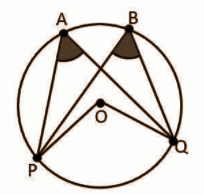A circle with centre O is given. Points P and Q on this circle subtends the angles ∠PAQ and ∠PBQ at points A and B, respectively.

To prove ∠PAQ = ∠PBQ

Proof:

Chord PQ subtends ∠POQ at the centre. From the theorem of “Angle subtended by an arc at the centre is double the angle subtended by it at any other point on the circle”.

∠POQ = 2∠PAQ —- (1)

∠POQ = 2∠PBQ —- (2)

From (1) and (2),

2∠PBQ = 2∠PAQ

∠PBQ = ∠PAQ

[ii]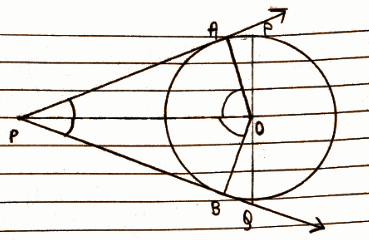In ΔAPO and ΔBPO

AP = BP (theorem)

OP = OP (common)

ΔAPO ≅ ΔBPO (by SSS congruence)

∠AOP = ∠BOP (hence, they subtend equal angle at centre P)

∠APO = ∠BPO (hence, they are equally inclined)

Question 10: Answer any one question [3 x 1 = 3]

[i] Two circles intersect each other at the points P and Q. If the diameters of the two circles are PA and PB respectively, then prove that A, Q, B are collinear.

[ii] ABC is a right-angled triangle whose ∠A = 90o, AD is perpendicular to BC. Prove that area of triangle ABC / area of the triangle ACD = BC2 / AC2.

Solution:

[i]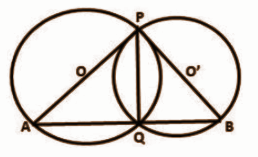Let O and O’ be the centres of two intersecting circles, where Points of intersection are P and Q and PA and PB are their diameter respectively.

Join PQ, AQ and QB.

∴ ∠AQP = 90° and ∠BQP = 90° (Angle in a semicircle is a right angle)

∠AQP + ∠BQP = 180°

∠AQB = 180°

Hence, the points A, Q and B are collinear.

[ii]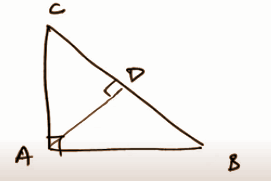In triangle ABC and ACD,

∠ACB = ∠ACD [common angle]

∠DAC = ∠ABC

Therefore, △ABC ⩭ △ACD [By AAA criterion]

Area of △ABC / area of △ACD = BC2 / AC2 [by the theorem, the area of congruent triangles = ratio of the square of their corresponding sides]

Question 11: Answer any one question [5 x 1 = 5]

[i] Draw the mean proportional of line segments of lengths 4 cm and 3 cm.

[ii] Draw a circle of radius 3 cm. Construct a tangent to the circle at a point A on the circle.

Solution:

[i] Given any two straight lines of length 4cm and 3cm it is possible to find a straight line of length 𝑐 such that 4:𝑐 = 𝑐:3.

Construction

• Let 𝐴𝐵 = 4cm and 𝐵𝐶 = 3cm be the two given straight lines.
• Let 𝐴𝐵 and 𝐵𝐶 be placed in a straight line and let the semicircle 𝐴𝐷𝐶 be placed on 𝐴𝐶.
• Let 𝐵𝐷 be drawn perpendicular to 𝐴𝐶.
• Then 𝐵𝐷 is the required mean proportional.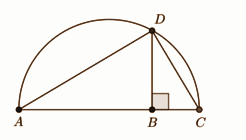[ii]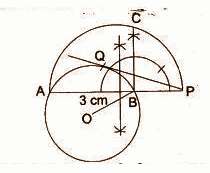1. Draw a circle with centre O and radius = 3cm.

2. Take any point P outside the circle.

3. Through the external point, P draws a straight line PBA meeting the circle at A and B.

4. Draw a semi-circle on AP as diameter.

5.Draw BC ⊥ AP, which intersects the semi-circle at C.

6. With centre P and radius, PC draws an arc cutting the circle at Q.

7. Join PQ.

Then PQ is the required tangent.

Question 12: Answer any two questions [3 x 2 = 6]

[i] If sin 17o = x / y, show that sec 17o – sin 73o = x2 / y√y2 – x2.

[ii] If the sum of two angles is 135o and their difference is 𝛑 / 12, then determine the sexagesimal and circular value of two angles.

[iii] Find the value of:

[5 cos2 (𝛑 / 3) + 4 sec2 (𝛑 / 6) – tan2 (𝛑 / 4)] / [sin2 (𝛑 / 6) + cos2 (𝛑 / 6)]

Solution:

[i] sin 17o = x / y

sec 17 – sin 73

1 / cos 17 – cos (90 – 17)

(1 – cos2 17) / cos 17

sin2 17 / (cos 17)2

sin2 17 / √1 – sin2 17

x2 / y2 / √1 – [x2 / y2]

x2 / y2 / √[y2 – x2] / y2

(x2 / y2) * (y / √y2 – x2]

x2 / y√y2 – x2

[ii] x + y = 135

x – y = 𝛑 / 12

x – y = 180 / 12

x + y = 135 —- (1)

x – y = 15 —- (1)

_________

2x = 150

x = 150 / 2

x = 75

Put the value of x in (1)

75 + y = 135

y = 135 – 75

y = 60

x = 75

= 75 * (𝛑 / 180)

= 5𝛑 / 12

y = 60

= 60 * (𝛑 / 180)

= 𝛑 / 3

[iii] [5 cos2 (𝛑 / 3) + 4 sec2 (𝛑 / 6) – tan2 (𝛑 / 4)] / [sin2 (𝛑 / 6) + cos2 (𝛑 / 6)]

= [5 cos2 (60) + 4 sec2 (30) – tan2 (45)] / [sin2 (30) + cos2 (30)]

= [5 * (0.52) + 4 * (1.33) – 1] / [0.52 + 0.75]

= 5.57 / 1

= 5.57

= 67 / 12

Question 13: Answer any one question [5 x 1 = 5]

[i] If the angle of elevation of a cloud from a point h metres above a lake is ɑ and the angle of depression of its reflection in the lake is β. Prove that the distance of the cloud from the point of observation is 2h sec ɑ / tan β – tan ɑ.

[ii] The heights of two towers are 180 metres and 60 metres respectively. If the angle of elevation of the top of the first tower from the foot of the second tower is 60o, then find the angle of elevation of the top of the second tower from the foot of the first.

Solution:

[i] Let the height of cloud from point of observation = C

Height of cloud from lake = C + h

Depth of cloud reflection from point of observation = C + h + h = C + 2h

Horizontal distance of cloud from point of observation = B

tan α = C / B

=> C = B tan α

tan β = (C + 2h) / B

=> C = B tan β – 2 h

B tan α = B tan β – 2 h

=> 2h = B tan β – B tan α

=> 2h = B (tan β – tan α)

=> B = 2h / (tan β – tan α)

cos α = B / distance of the cloud from the point of observation

=> distance of the cloud from the point of observation = B / cos α

= B sec α

= 2h sec α / (tan β – tan α)

The distance of the cloud from the point of observation = 2h sec α / (tan β – tan α).

[ii] Height of first tower (AB) = 180 m

Height of the second tower (DC) = 60 m

The angle of elevation of the top of the first tower from the foot of the second tower [∠ACB = 60°]

The angle of elevation of the top of the second tower from the foot of the first.

Suppose, the angle of elevation of the top of the second tower from the foot of the first [∠CBD] = ∅

∆ABC is a right triangle

tan 60° = AB / BC

→ √3 = 180 / BC

→ BC = 60√3

∆BCD is a right triangle.

tan ∅ = DC / BC

→ tan ∅ = 60 / 60√3

→ tan ∅ = 1 / √3

→ tan ∅ = tan30°

→ ∅ = 30°

The angle of elevation of the top of the second tower from the foot of the first is 30°.

Question 14: Answer any two questions [4 x 2 = 8]

[i] The length of outer and inner radii of a hollow right circular pipe is 5 cm 4 cm respectively. If the total surface area of the pipe is 1188 sq. cm. find the length of the pipe.

[ii] A hemisphere pot with an internal radius of 9 cm is completely filled with water in cylindrical bottles with a diameter of 3 cm and height of 4 cm, then find the number of bottles to be required to make the pot empty.

[iii] The diameter of the base of a right circular cone is 21 metres and height is 14 metres. What will be the expenditure to colour the curved surface at the rate of Rs 1.50 per square metre?

Solution:

[i] 2𝛑 (r1 + r2) h + 2𝛑 (r12 – r22) = 1188

𝛑 ((5 + 4)h + (52 – 42)) = 1188 / 2

(22 / 7) [9h + 9] = 594

9h + 9 = 189

9h = 189 – 9

9h = 180

h = 180 / 9

h = 20cm

[ii] Volume of a hemisphere = 2𝛑r3 / 3

= 2 * (22 / 7) * (93) / 3

= 4582.3 / 3

= 1527.3

Volume of the cylinder = 𝛑r2h

= (22 / 7) * (3 / 2)2 * 4

= 28.29

Number of bottles = 1527.3 / 28.3

= 54

[iii] Let, the base radius of the cone = r m

According to the problem,

⇒ πr2 = 21

⇒ r2 = 21 × 7 / 22

⇒ r = 2.58

∴ The radius of the base = 2.58 m

Height of the cone = 14 m

∴ The slant height of the cone,

= √(2.582 + 142)

= √(6.66 + 196)

= 14.24 cm

∴ The curved surface area of the cone,

= πrl

= 22 / 7 × 2.58 × 14.24

= 115.47 sq. m

∴ Expenditure to colour the curved surface area,

= 115.47 × 1.50

= Rs. 173.2

Question 15: Answer any two questions [4 x 2 = 8]

[i] Find the mean of marks obtained by the girl students if their cumulative frequencies are as follows:

 Marks <10 <20 <30 <40 <50 Number of girl students 6 10 18 30 46

[ii] Find the median of data from the following frequency distribution table:

 Class interval 0 – 10 10 – 20 20 – 30 30 – 40 40 – 50 50 – 60 60 – 70 Frequency 4 7 10 15 10 8 5

[iii] Find the mode of data from the following frequency distribution table.

 Class 3 – 6 6 – 9 9 – 12 12 – 15 15 – 18 18 – 21 21 – 24 Frequency 2 6 12 24 21 12 3

Solution:

[i]
 Marks Number of girl students Marks Frequency Mid value xifi <10 6 0 – 10 6 5 30 <20 10 10 – 20 4 15 60 <30 18 20 – 30 8 25 200 <40 30 30 – 40 12 35 420 <50 46 40 – 50 16 45 720

Mean = ∑xifi / ∑fi

= 1430 / 46

= 31.08

[ii]
 Class Frequency Cumulative frequency 0 – 10 4 4 10 – 20 7 11 20 – 30 10 21 30 – 40 15 36 40 – 50 10 46 50 – 60 8 54 60 – 70 5 59

Median = 59 + 1 / 2 = 30th term

Median = L + {(n + 1) / 2) – c.f.} * (h/f)

Median class = 20 – 30

= 20 + (30) – 11) * (10 / 10)

= 20 + 19 * 1

= 39

[iii] Modal class = 12 – 15

l = 12, f1 = 24, fo = 12, f2 = 21, h = 3

Z = l + (f1 – f0) / (2f1 – f0 – f2) * h

= 12 + (24 – 12) / (2 * 24 – 12 – 21) * 3

= 12 + 12 / 15 * 3

= 14.4

[Alternative Question for Sightless Candidates]

Question 11: Answer any one question [5 x 1 = 5]

[i] Describe the process of drawing the mean proportional of two line segments of a given length.

[ii] A circle is given, describe the process of drawing a tangent to this circle at a point on it.

Solution:

[i] Given any two straight lines of length a and b it is possible to find a straight line of length 𝑐 such that a:𝑐 = b:3.

Construction

• Let a and b be the two given straight lines.
• Let 𝐴𝐵 and 𝐵𝐶 be placed in a straight line and let the semicircle 𝐴𝐷𝐶 be placed on 𝐴𝐶.
• Let 𝐵𝐷 be drawn perpendicular to 𝐴𝐶.
• Then 𝐵𝐷 is the required mean proportional.[ii] Construction of a tangent to a circle

To draw a tangent to a circle at a point on the circle. (Refer fig.)

Step 1: Draw a circle with the required radius with centre O.

Step 2: Join centre of the circle O and any point P on the circle. OP is the radius of the circle.

Step 3: Draw a line perpendicular to radius OP through point P. This line will be a tangent to the circle at P.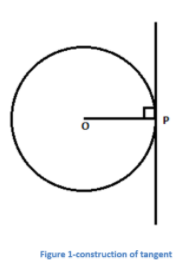Question 16: (a) Answer any three questions [2 x 3 = 6]

[i] If the percentage of profit on the sale price is 20%, then what are the percentages of profit on the cost price?

[ii] If x = 3cosθ ; y = 3sinθ, then find the value of x2 + y2.

[iii] Simplify: √98 + √8 – 2 √32.

[iv] The ratio of the lengths of the radii of the bases a right circular cylinder and a right circular cone 3:4 and the ratio of their heights are 2:3; what is the ratio of their volume?

Solution:

[i] 20% of profits on sales mean 25% profits to cost. Thus the cost will be 75, profit will be 25 if the sales will be 100.

[ii] x = 3cosθ ; y = 3sinθ

x2 + y2 = (3 cos θ)2 + (3 sin θ)2

= 9 cos2 θ + 9 sin2 θ

= 9 (cos2 θ + sin2 θ)

= 9

[iii] √98 + √8 – 2 √32

= √49 * 2 + √4 * 2 – 2 √16 * 2

= 7√2 + 2√2 – 8√2

= √2

[iv] Volume of the cone = (1 / 3) 𝛑r12h1

The volume of the cylinder = 𝛑r22h2

r2 / r1 = 3 / 4

h2 / h1 = 2 / 3

Volume of the cylinder / Volume fo the cone = 𝛑r22h2 / ((1 / 3) 𝛑r12h1)

= 3 [r2 / r1]2 [h2 / h1]

= 3 * (9 / 16) * (2 / 3)

= 9 / 8

= 9:8

(b) Answer any four questions [1 x 4 = 4]

[i] Find the number of years for which a principal becomes double at the rate of simple interest of 6 (1 / 4) % per annum.

[ii] AB is a diameter of a circle and P is any point on the circle if PAB = 30o find the value of the angle PBA.

[iii] Express 22o 30’ in radian.

[iv] If the radius of a solid sphere is 10.5 cm, then what is the area of its whole surface?

[v] If x : y = 2 : 3 and y : z = 4 : 7, then find x : z.

Solution:

[i] Let the principal amount be P, Simple interest amount is also P (This is because the money gets doubled if half the doubled amount will be the principal amount then the remaining amount will be its interest). Hence, the interest and the principal amount is equal here.

Let the number of years be “T”.

Therefore,

A = PTR / 100

2P = [P * T * 6.25] / 100

200P = 6.25PT

200P / 6.25P = T

T = 32 years

[ii] ⇒ ∠PAB = 30o

⇒ ∠BPA = 90o [angle inscribed in a semi-circle]

⇒ ∠PAB + ∠PBA + ∠BPA = 180o

∴ 30o + ∠PBA + 90o = 180o

∴ ∠PBA = 180o − 120o

∴ ∠PBA = 60o

[iii] 22 30′

= (20 + 30)

= (20 + 30 / 60)

= 45 / 2

= [45 / 2] * [π / 180]

= π / 8

[iv] r = 10.5 cm

The surface area of sphere = 4πr2

​= 4 * (22 / 7) * (10.5)2

= 1386 cm2

[v] x : y = 2 : 3 and y : z = 4 : 7

x : z = ?

x / y = 2 / 3

x = 2y / 3

y / z = 4 / 7

7y = 4z

z = 7y / 4

x / z = (2y / 3) / (7y / 4)

x : z = 8 : 21